# 数据结构与算法复习（持续更新中）

1.数组实现（自动扩容）

2.链表实现

1.栈在函数调用中的应用

2.栈在表达式求值中的应用（比如：34+13*9+44-12/3）

3.栈在括号匹配中的应用（比如：{}{[()]()}）

4.如何实现浏览器的前进后退功能？

O(n)时间复杂度内求无序数组中的第K大元素。

为什么归并排序并不常用

Glibc中的qsort()函数

O(logn)惊人的查找速度

LRU缓存淘汰算法

# 栈

## 函数调用栈来保存临时变量，为什么函数调用要用“栈”来保存临时变量呢？用其他数据结构不行吗？

### 什么是栈？

1. 后进者先出，先进者后出，这就是典型的结构。
2. 从栈的操作特性来看，是一种操作受限的线性表，只允许在端插入和删除数据。

### 为什么需要栈？

1. 栈是一种操作受限的数据结构，其操作特性用数组和链表均可实现。
2. 但，任何数据结构都是对特定应用场景的抽象，数组和链表虽然使用起来更加灵活，但却暴露了几乎所有的操作，难免会引发错误操作的风险。
3. 所以，当某个数据集合只涉及在某端插入和删除数据，且满足后进者先出，先进者后出的操作特性时，我们应该首选栈这种数据结构。

# 队列

## 优化：出队时可以不用搬移数据。如果没有空闲空间了，我们只需要在入队时，再集中触发一次数据的搬移操作。

// 入队操作，将item放入队尾
public boolean enqueue(String item) {
// tail == n表示队列末尾没有空间了
if (tail == n) {
if (head == 0) return false;
// 数据搬移
for (int i = head; i < tail; ++i) {
}
}

items[tail] = item;
++tail;
return true;

# 递归

## 递归需要满足的三个条件

1. 一个问题的解可以分解为几个子问题的解
2. 这个问题与分解之后的子问题，除了数据规模不同，求解思路完全一样
3. 存在递归终止条件

## 如何避免出现堆栈溢出

// 全局变量，表示递归的深度。
int depth = 0;
int f(int n) {
++depth；
if (depth > 1000) throw exception;

if (n == 1) return 1;
return f(n-1) + 1;
}

1. 打印日志发现，递归值。
2. 结合条件断点进行调试。

# 排序

## 如何分析一个“排序算法”？

### 排序算法的执行效率

1. 最好情况、最坏情况、平均情况时间复杂度

2. 时间复杂度的系数、常数 、低阶

3. 比较次数和交换（或移动）次数

## 冒泡排序（Bubble Sort）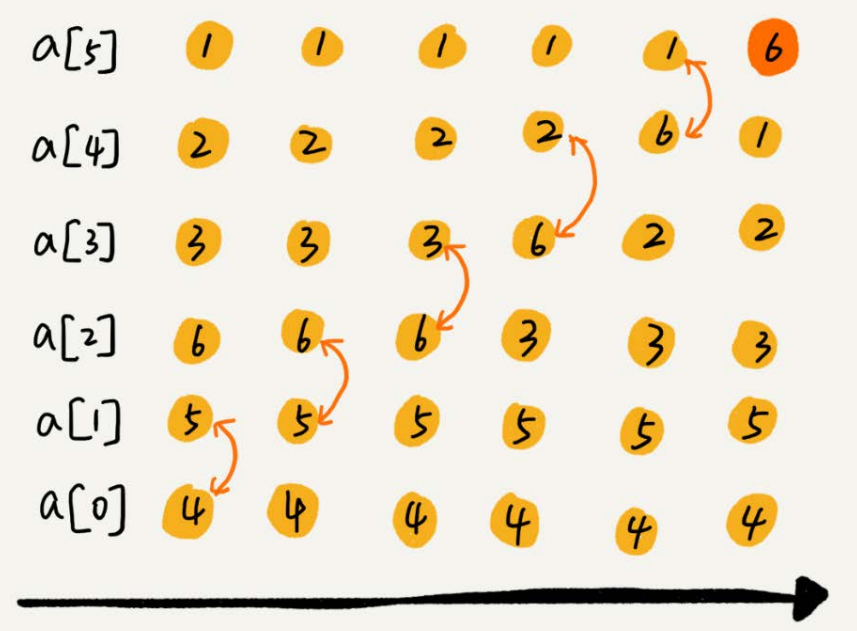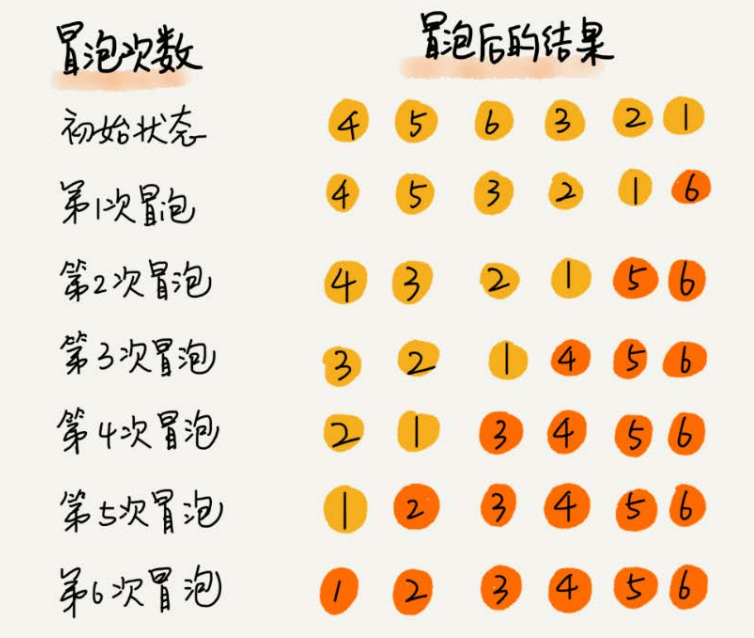### 有序度

// 冒泡排序，a表示数组，n表示数组大小
public void bubbleSort(int[] a, int n) {
if (n <= 1) return;
for (int i = 0; i < n; ++i) {
// 提前退出冒泡循环的标志位
boolean flag = false;
for (int j = 0; j < n - i - 1; ++j) {
if (a[j] > a[j+1]) { // 交换
int tmp = a[j];
a[j] = a[j+1];
a[j+1] = tmp;
flag = true; // 表示有数据交换
}
}
if (!flag) break; // 没有数据交换，提前退出
}
}


## 插入排序（Insertion Sort）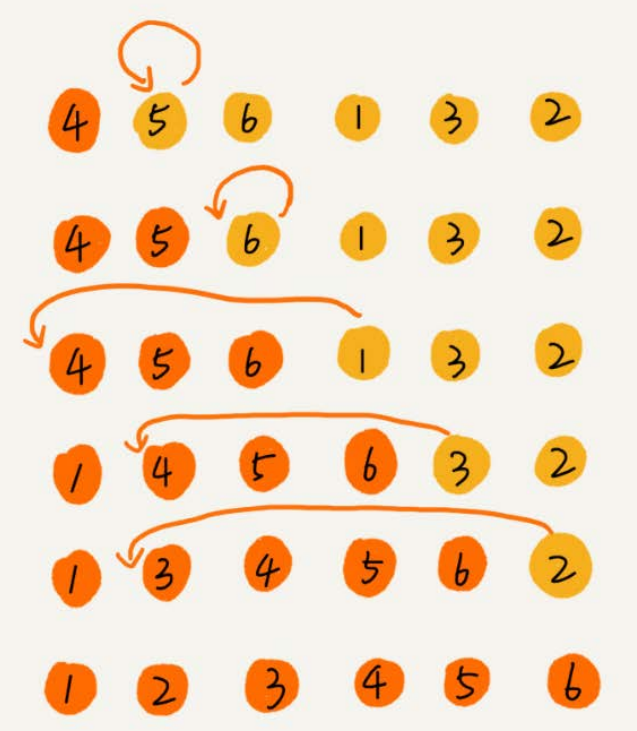// 插入排序，a表示数组，n表示数组大小
public void insertionSort(int[] a, int n) {
if (n <= 1) return;
for (int i = 1; i < n; ++i) {
int value = a[i];
int j = i - 1;
// 查找插入的位置
for (; j >= 0; --j) {
if (a[j] > value) {
a[j+1] = a[j]; // 数据移动
}
else {
break;
}
}
a[j+1] = value; // 插入数据
}
}

## 选择排序（Selection Sort）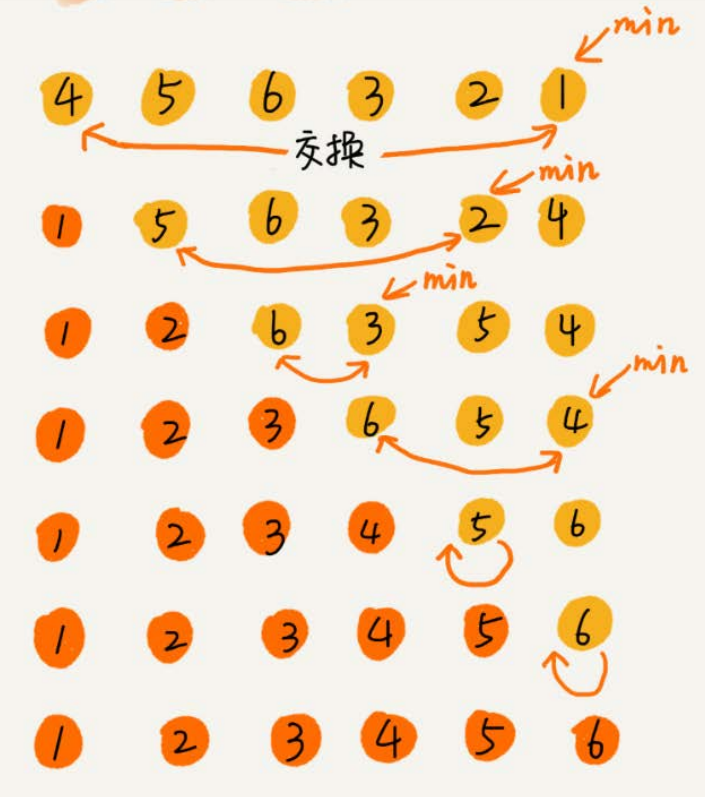### 冒泡排序和插入排序的时间复杂度都是O(n2)，都是原地排序算法，为什么插入排序要比冒泡排序更受欢迎呢？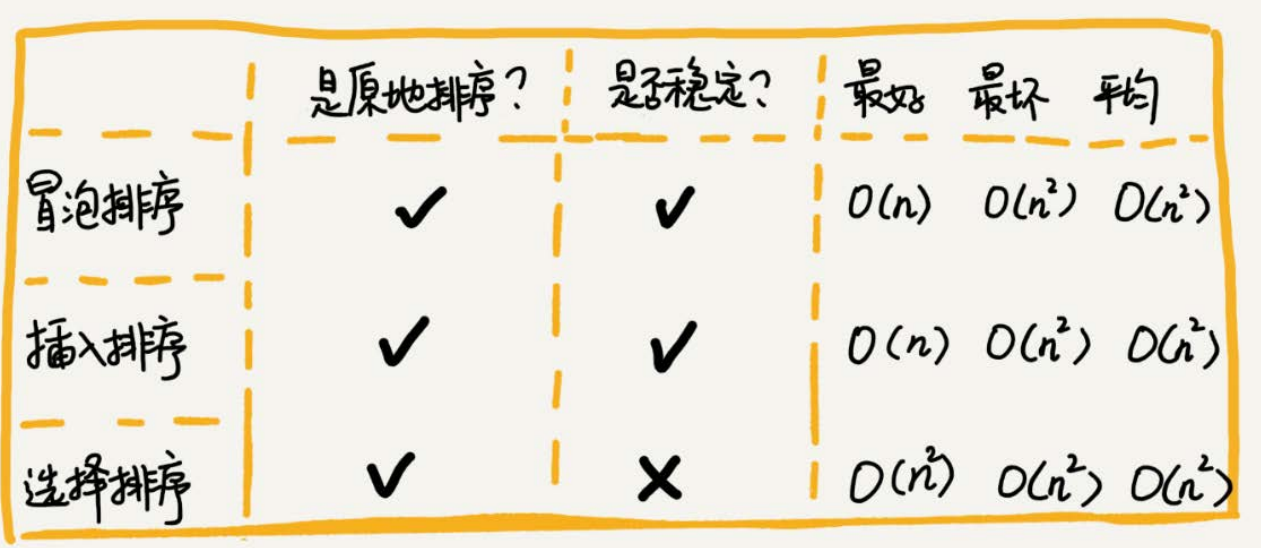## 归并排序（Merge Sort）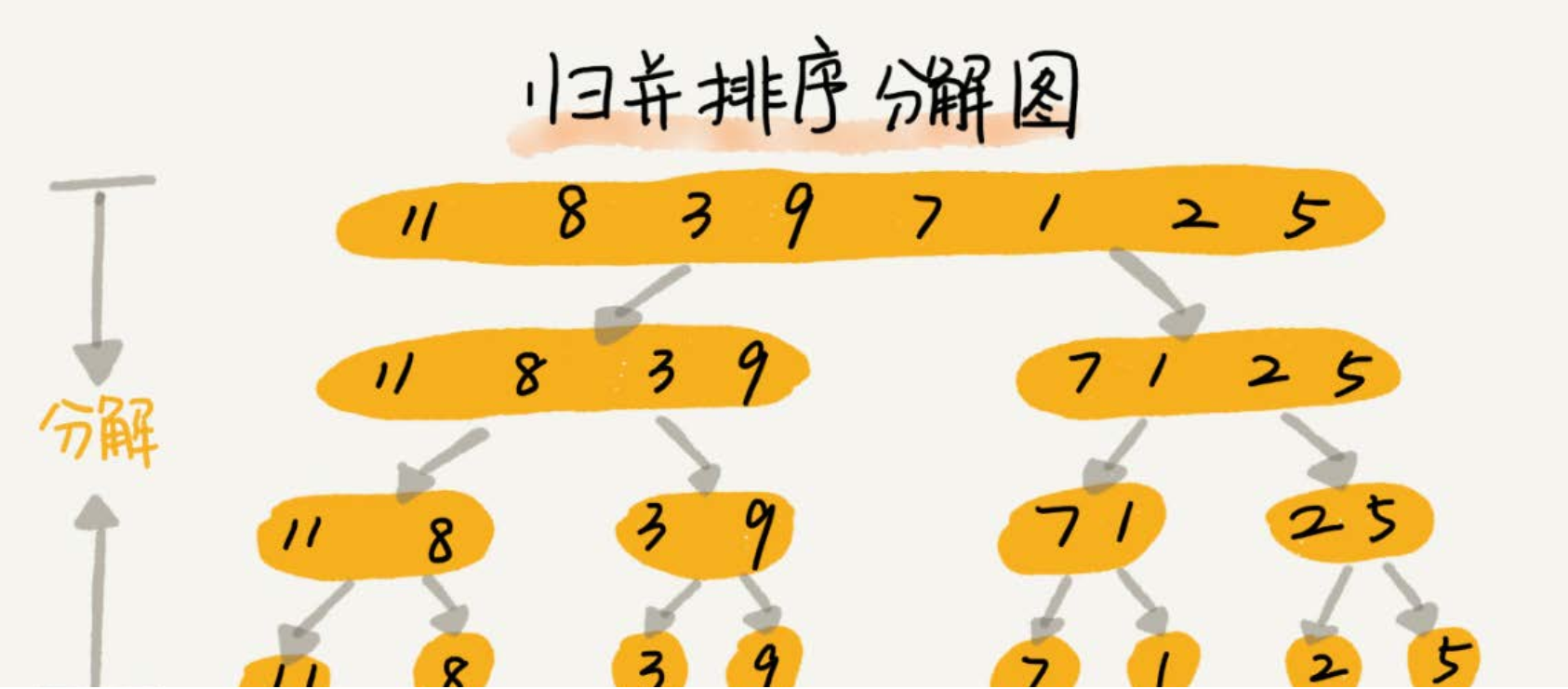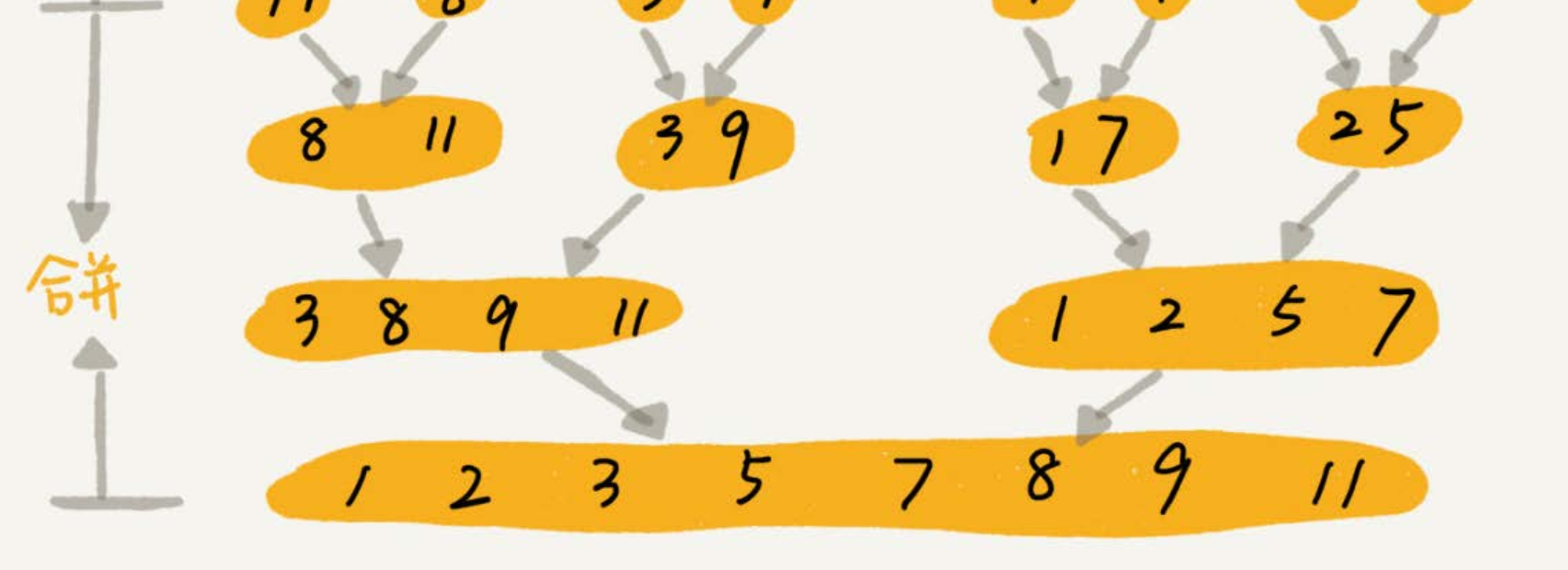// 归并排序算法, A是数组，n表示数组大小
merge_sort(A, n) {
merge_sort_c(A, 0, n-1)
}
// 递归调用函数
merge_sort_c(A, p, r) {
// 递归终止条件
if p >= r then return
// 取p到r之间的中间位置q
q = (p+r) / 2
// 分治递归
merge_sort_c(A, p, q)
merge_sort_c(A, q+1, r)
// 将A[p...q]和A[q+1...r]合并为A[p...r]
merge(A[p...r], A[p...q], A[q+1...r])
}

merge(A[p…r], A[p…q], A[q+1…r])这个函数的作用就是，将已经有序的A[p…q]和A[q+1…r]合并成一个有序的数组，并且放入A[p…r]。那这过程具体该如何做呢？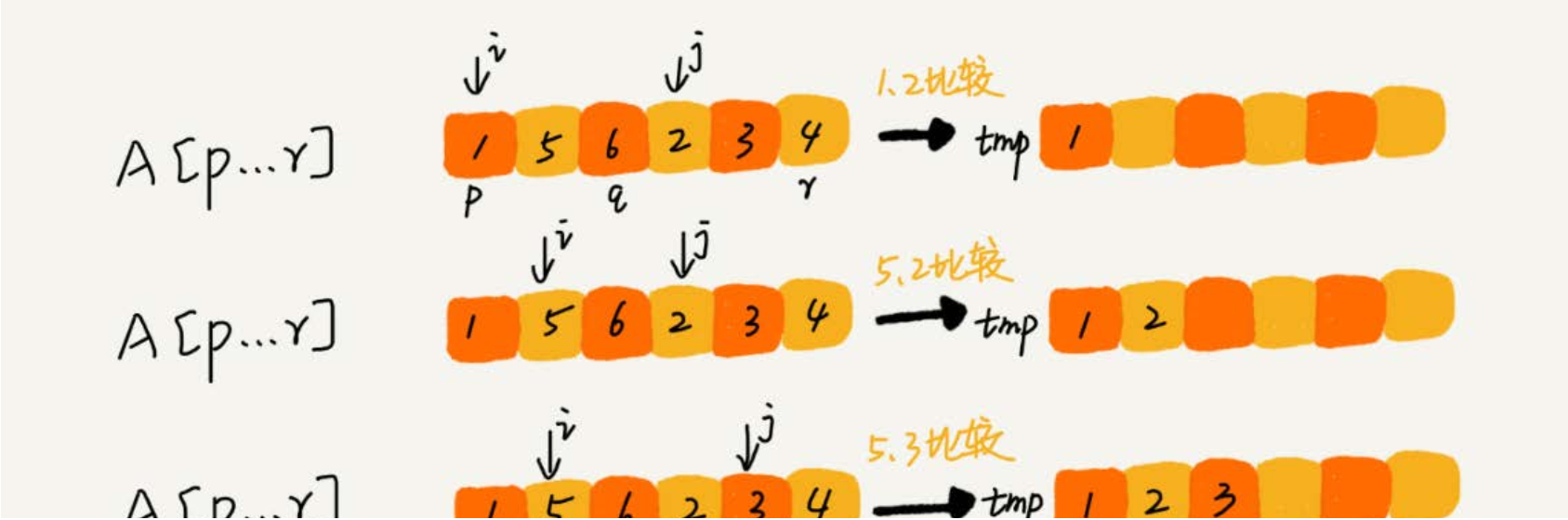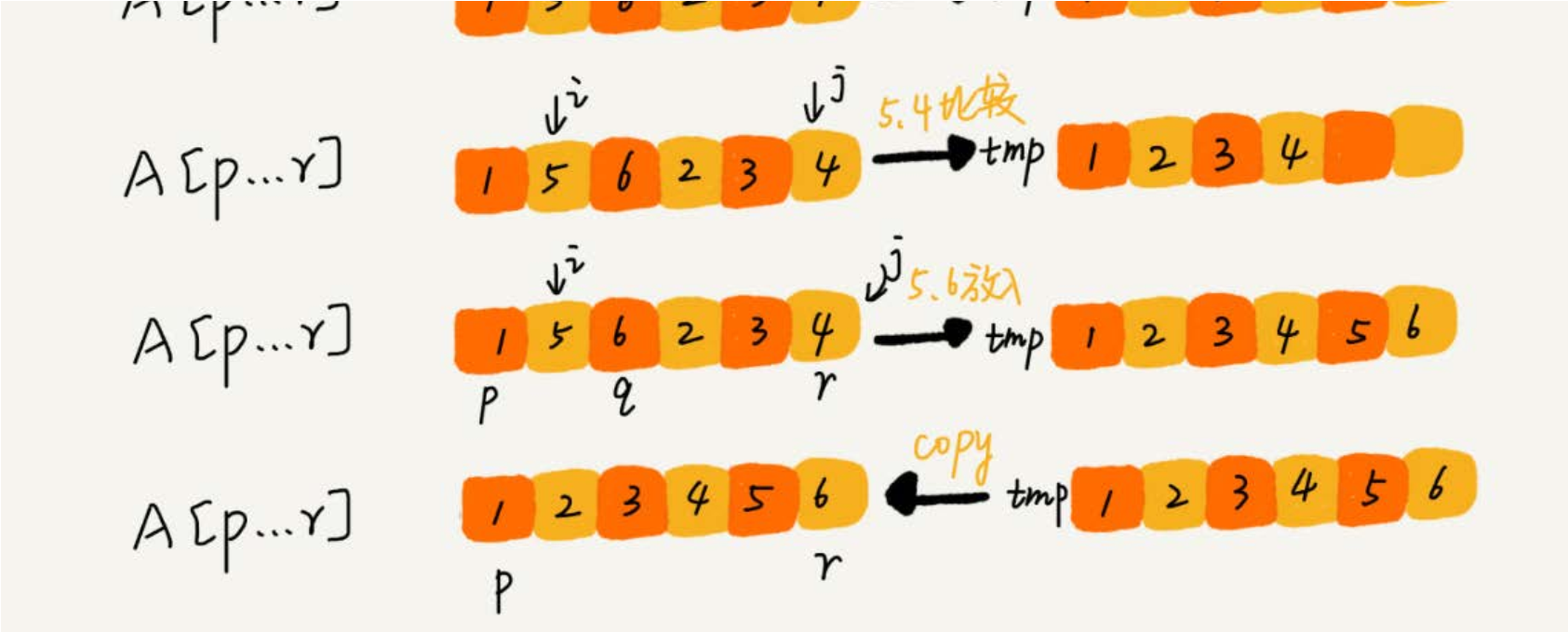merge(A[p...r], A[p...q], A[q+1...r]) {
var i := p，j := q+1，k := 0 //初始化变量i, j, k
var tmp := new array[0...r-p] //申请一个大小跟A[p...r]一样的临时数组
while i<=q AND j<=r do {
if A[i] <= A[j] {
tmp[k++] = A[i++] // i++等于i:=i+1
} else {
tmp[k++] = A[j++]
}
}
//判断哪个子数组中有剩余的数据
var start := i，end := q
if j<=r then start := j, end:=r
//将剩余的数据拷贝到临时数组tmp
while start <= end do {
tmp[k++] = A[start++]
}
//将tmp中的数组拷贝回A[p...r]
for i:=0 to r-p do {
A[p+i] = tmp[i]
}
}

### 归并排序的性能分析

T(1) = C；n=1时，只需要常量级的执行时间，所以表示为C。
T(n) = 2*T(n/2) + n；n>1

T(n) = 2*T(n/2) + n
= 2*(2*T(n/4) + n/2) + n = 4*T(n/4) + 2*n
= 4*(2*T(n/8) + n/4) + 2*n = 8*T(n/8) + 3*n
= 8*(2*T(n/16) + n/8) + 3*n = 16*T(n/16) + 4*n
......
= 2^k * T(n/2^k) + k * n

## 快速排序算法（Quicksort）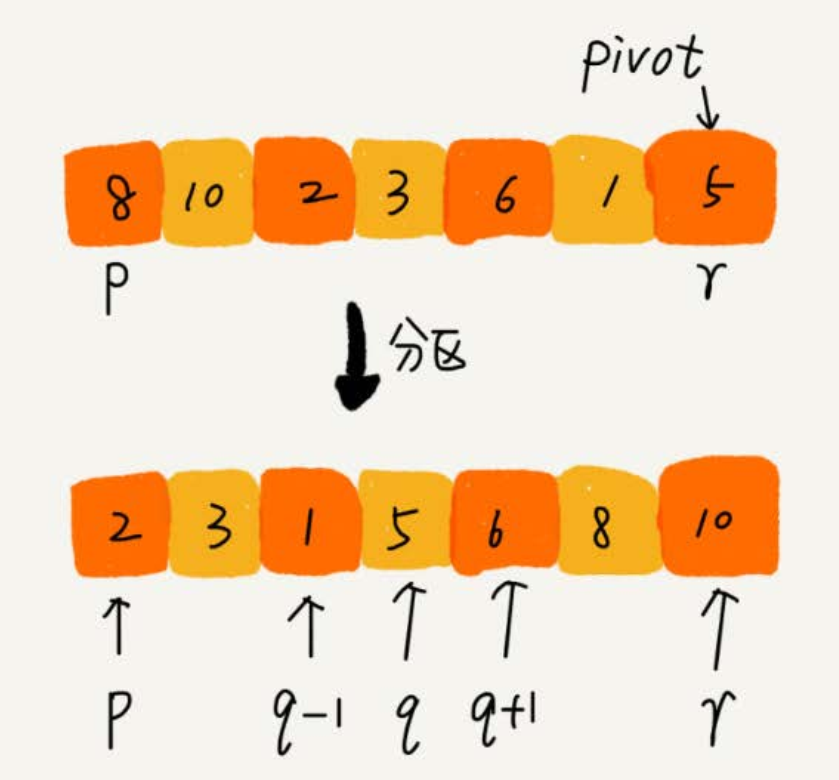quick_sort(p…r) = quick_sort(p…q-1) + quick_sort(q+1, r)

p >= r

//快速排序，A是数组，n表示数组的大小
quick_sort(A, n) {
quick_sort_c(A, 0, n-1)
}
//快速排序递归函数，p,r为下标
quick_sort_c(A, p, r) {
if p >= r then return
q = partition(A, p, r) //获取分区点
quick_sort_c(A, p, q-1)
quick_sort_c(A, q+1, r)
}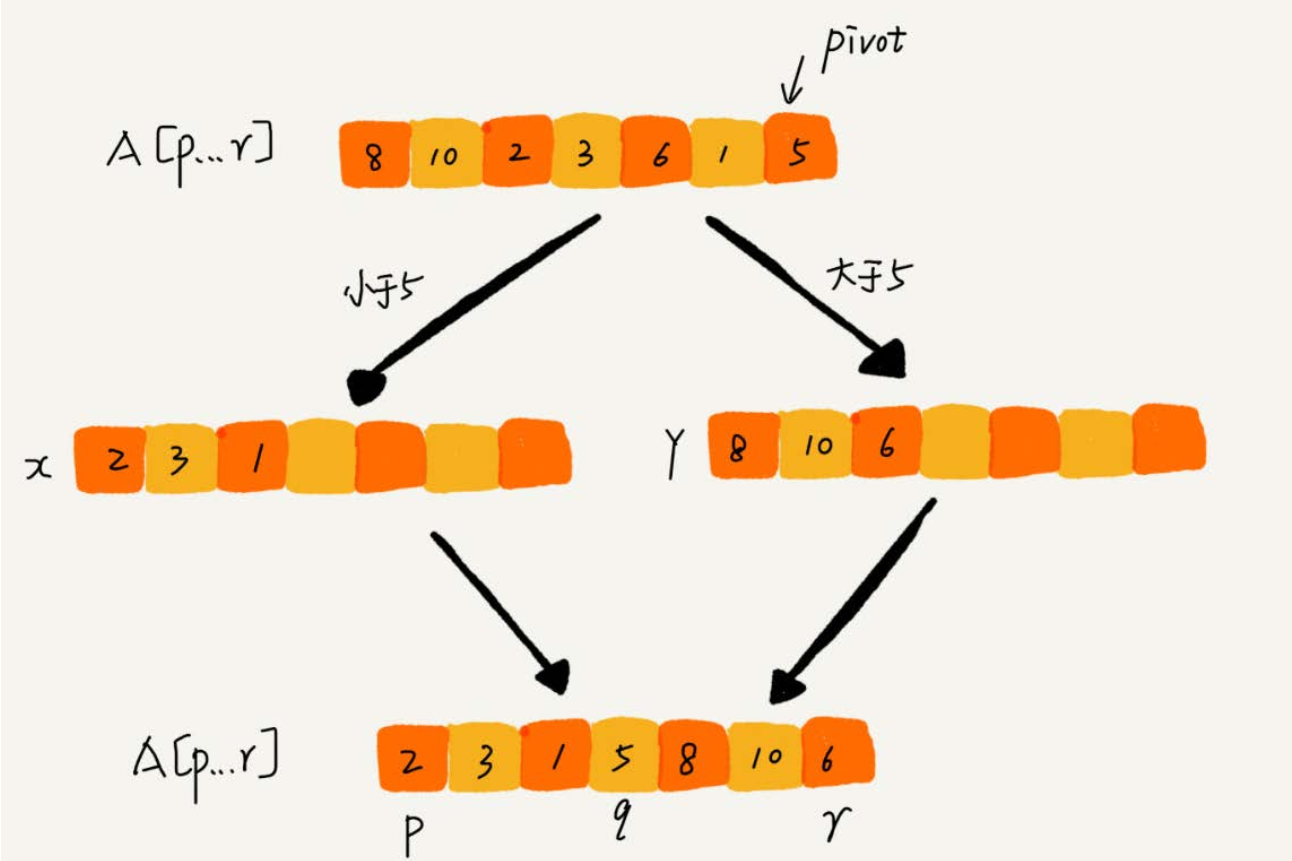partition(A, p, r) {
pivot := A[r]
i := p
for j := p to r-1 do {
if A[j] < pivot {
swap A[i] with A[j]
i := i+1
}
}
swap A[i] with A[r]
return i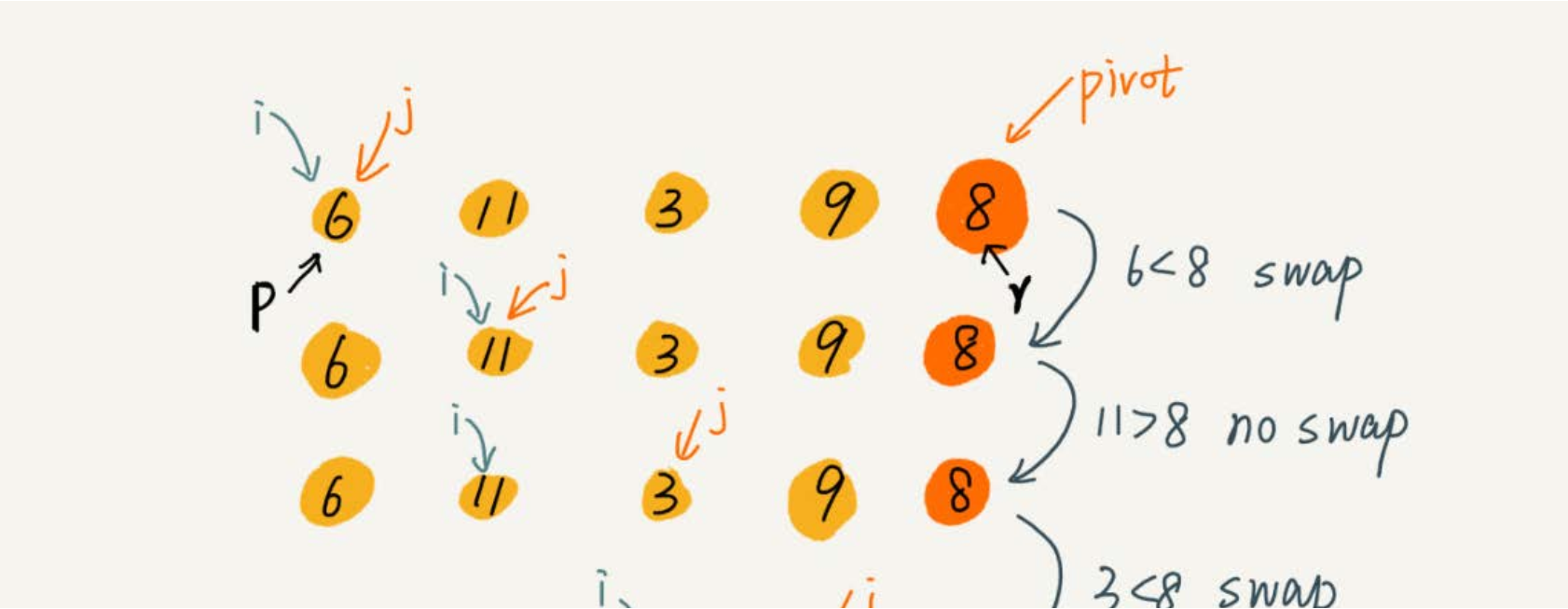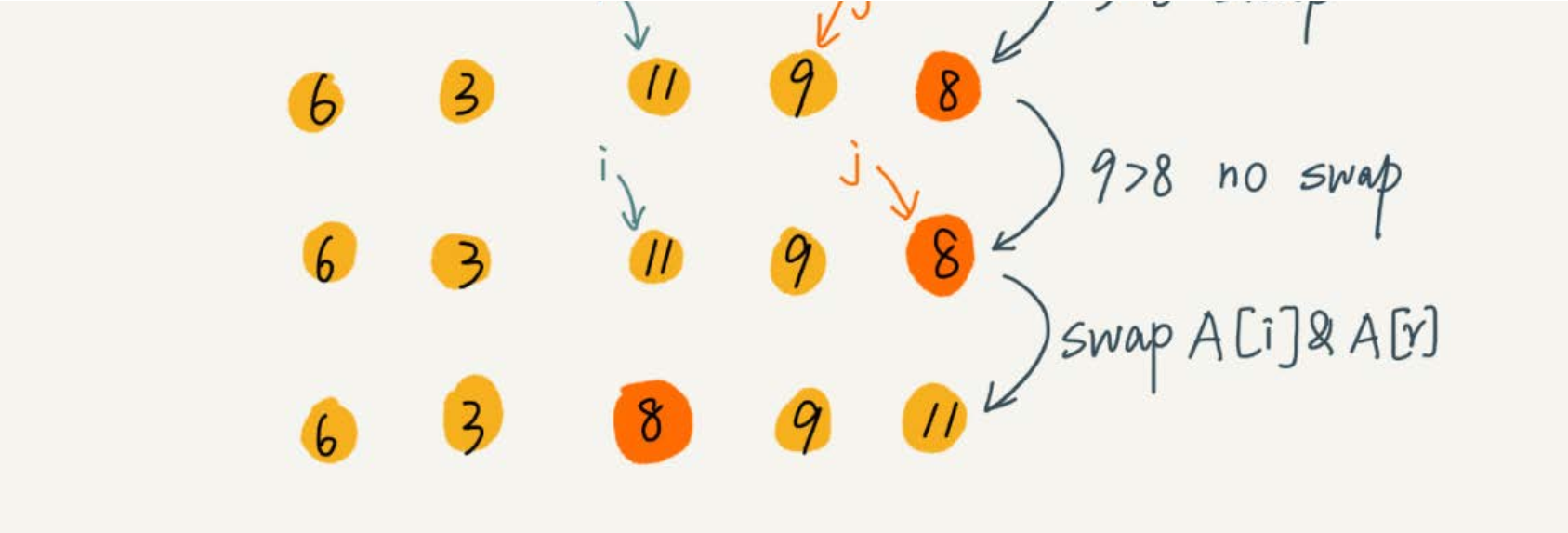j++;        if(swap) i++;

### 快排和归并的区别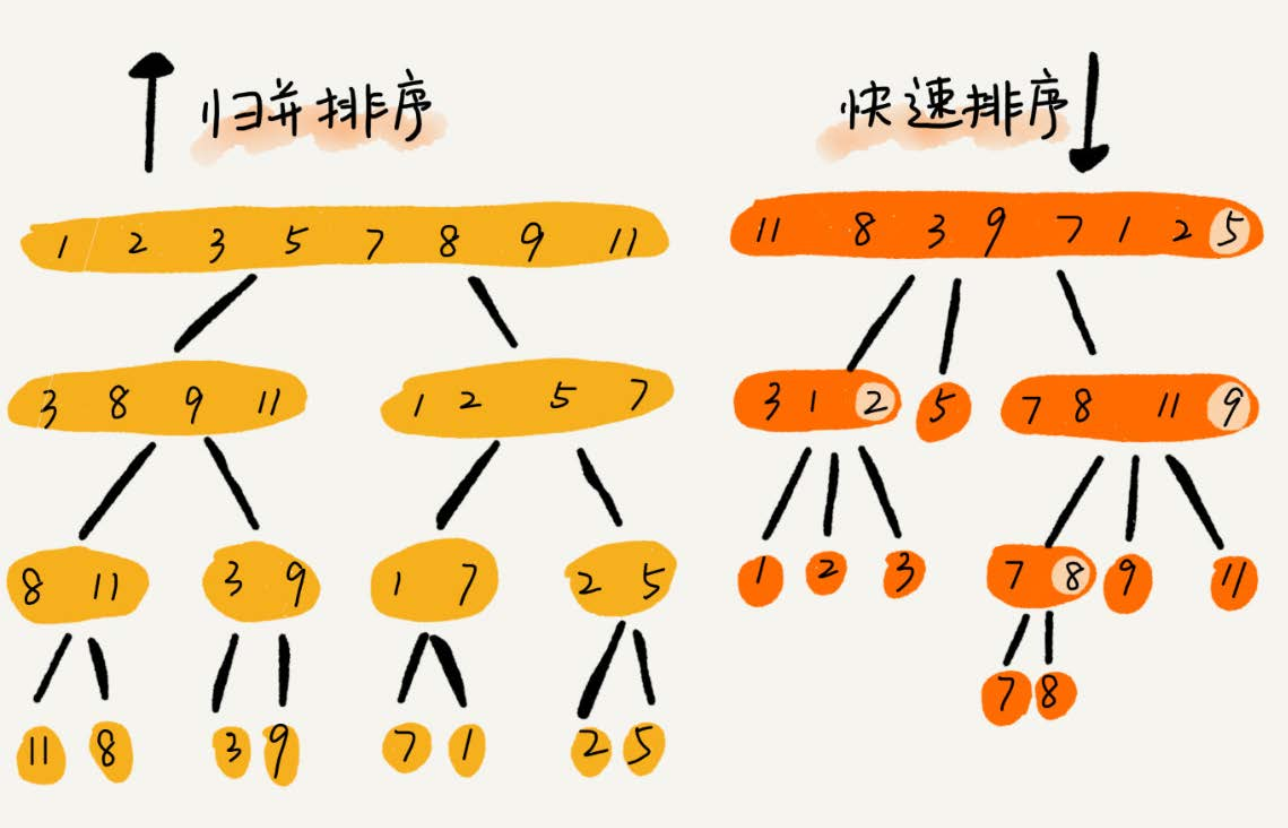## 计数排序（Counting sort）

### 我们都经历过高考，高考查分数系统你还记得吗？我们查分数的时候，系统会显示我们的成绩以及所在省的排名。如果你所在的省有50万考生，如何通过成绩快速排序得出名次呢？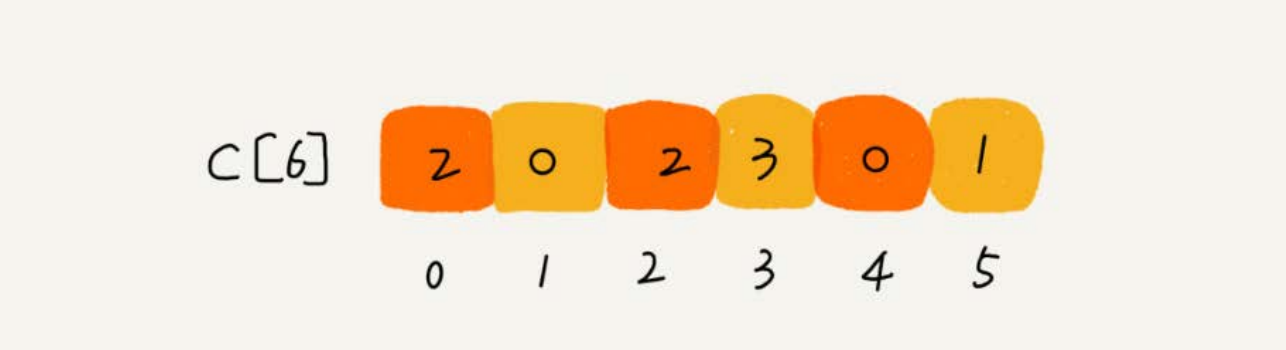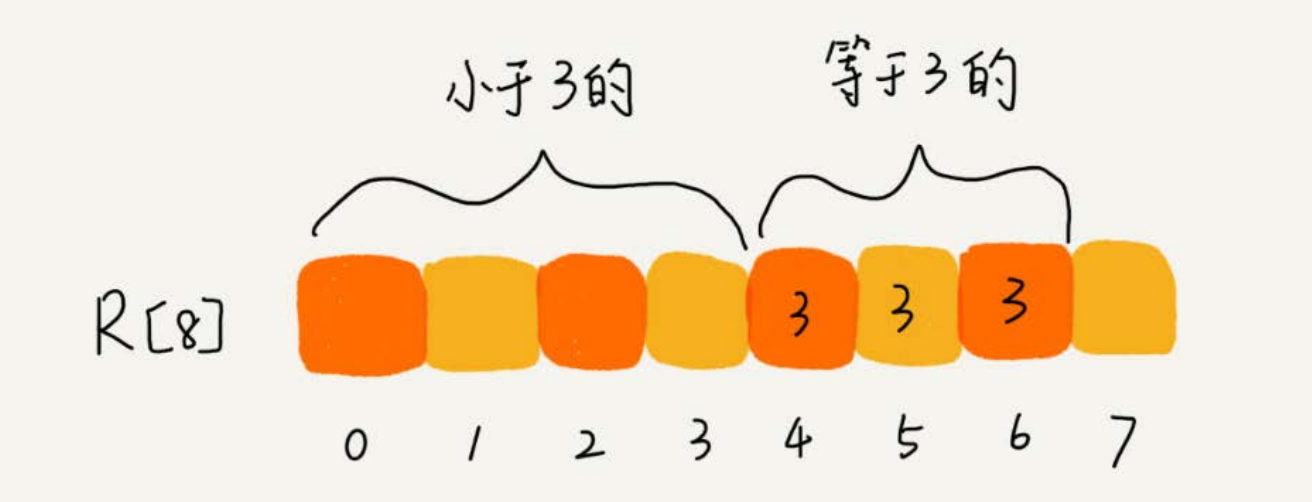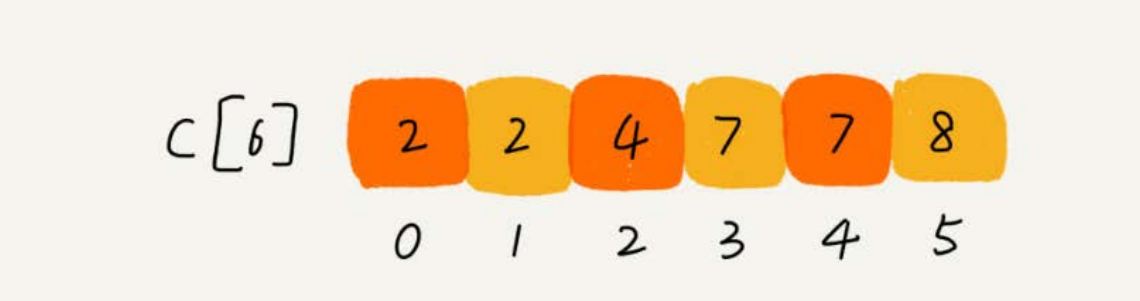// 计数排序，a是数组，n是数组大小。假设数组中存储的都是非负整数。
public void countingSort(int[] a, int n) {
if (n <= 1) return;
// 查找数组中数据的范围
int max = a;
for (int i = 1; i < n; ++i) {
if (max < a[i]) {
max = a[i];
}
}
int[] c = new int[max + 1]; // 申请一个计数数组c，下标大小[0,max]
for (int i = 0; i <= max; ++i) {
c[i] = 0;
}
// 计算每个元素的个数，放入c中
for (int i = 0; i < n; ++i) {
c[a[i]]++;
}
// 依次累加
for (int i = 1; i <= max; ++i) {
c[i] = c[i-1] + c[i];
}
// 临时数组r，存储排序之后的结果
int[] r = new int[n];
// 计算排序的关键步骤，有点难理解
for (int i = n - 1; i >= 0; --i) {
int index = c[a[i]]-1;
r[index] = a[i];
c[a[i]]--;
}
// 将结果拷贝给a数组
for (int i = 0; i < n; ++i) {
a[i] = r[i];
}
}

### 假设我们有10万个手机号码，希望将这10万个手机号码从小到大排序，你有什么比较快速的排序方法呢？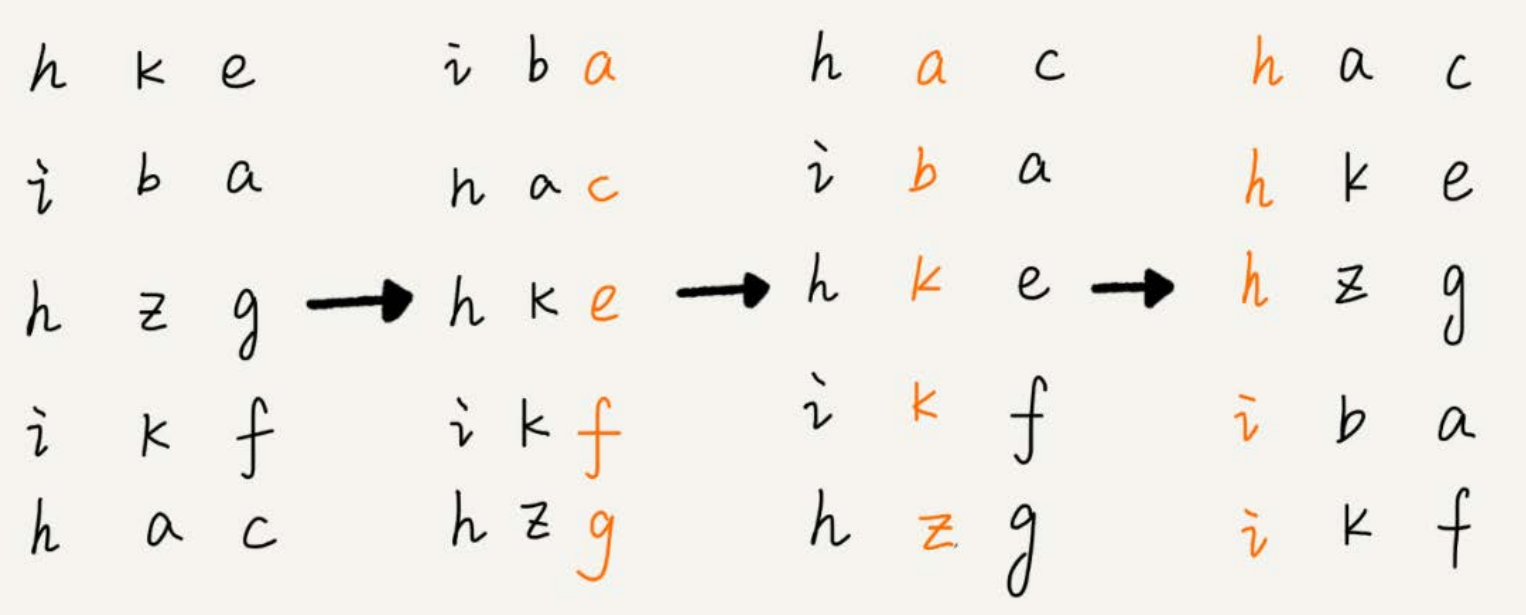## 如何选择合适的排序算法？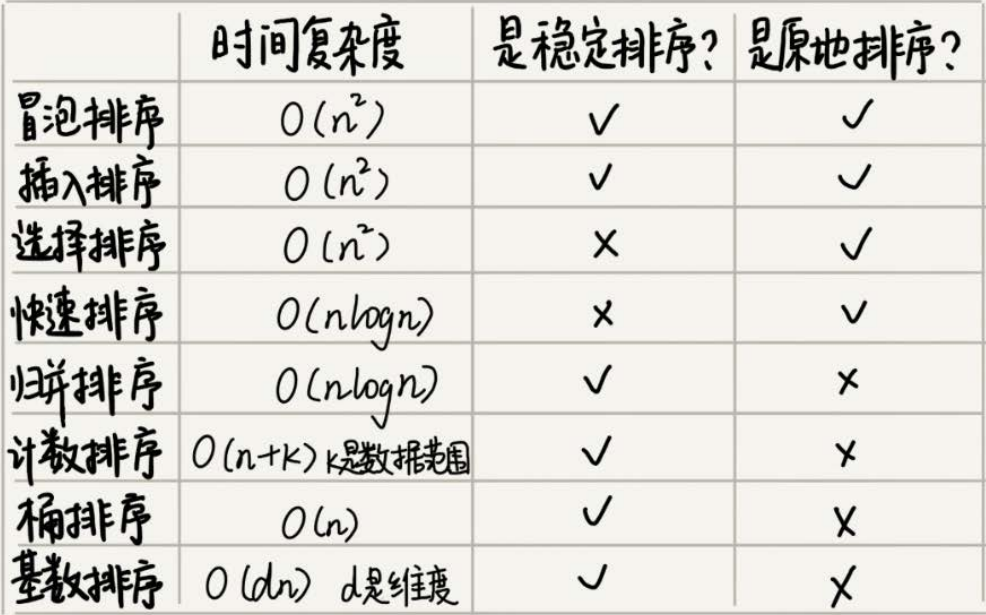1.三数取中法

2.随机法

### Glibc中的qsort()函数

qsort()优先使用归并排序来排序输入数据，因为归并排序的空间复杂度是O(n)，所以对于小数据量的排序，比如1KB、2KB等，归并排序额外需要1KB、2KB的内存空间。现在计算机的内存都很大，很多时候追求的是速度，这是典型的用空间换时间技巧的应用。

knlogn+c = 1000 * 100 * log100 + 200 远大于10000

n^2 = 100*100 = 10000

## 堆和堆排序

）的应用场景非常多，最经典的莫过于堆排序了。堆排序是一种原地的、时间复杂度为 的排序算法。

• 堆是一个完全二叉树；
• 堆中每一个节点的值都必须大于等于（或小于等于）其子树中每个节点的值。

### 如何实现一个堆？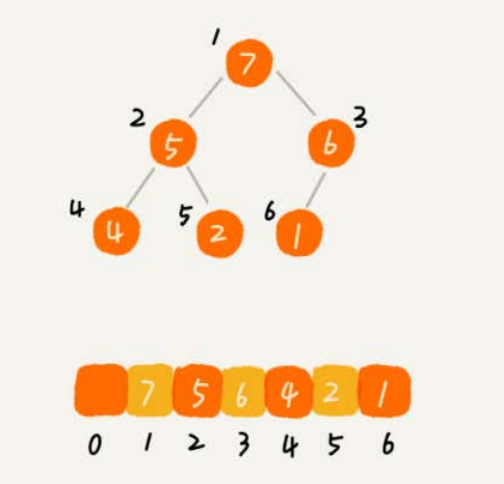### 1.往堆中插入一个元素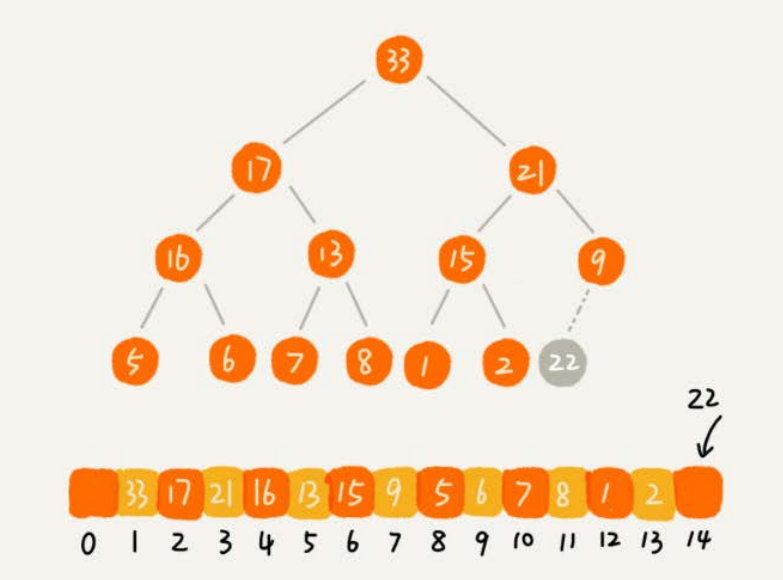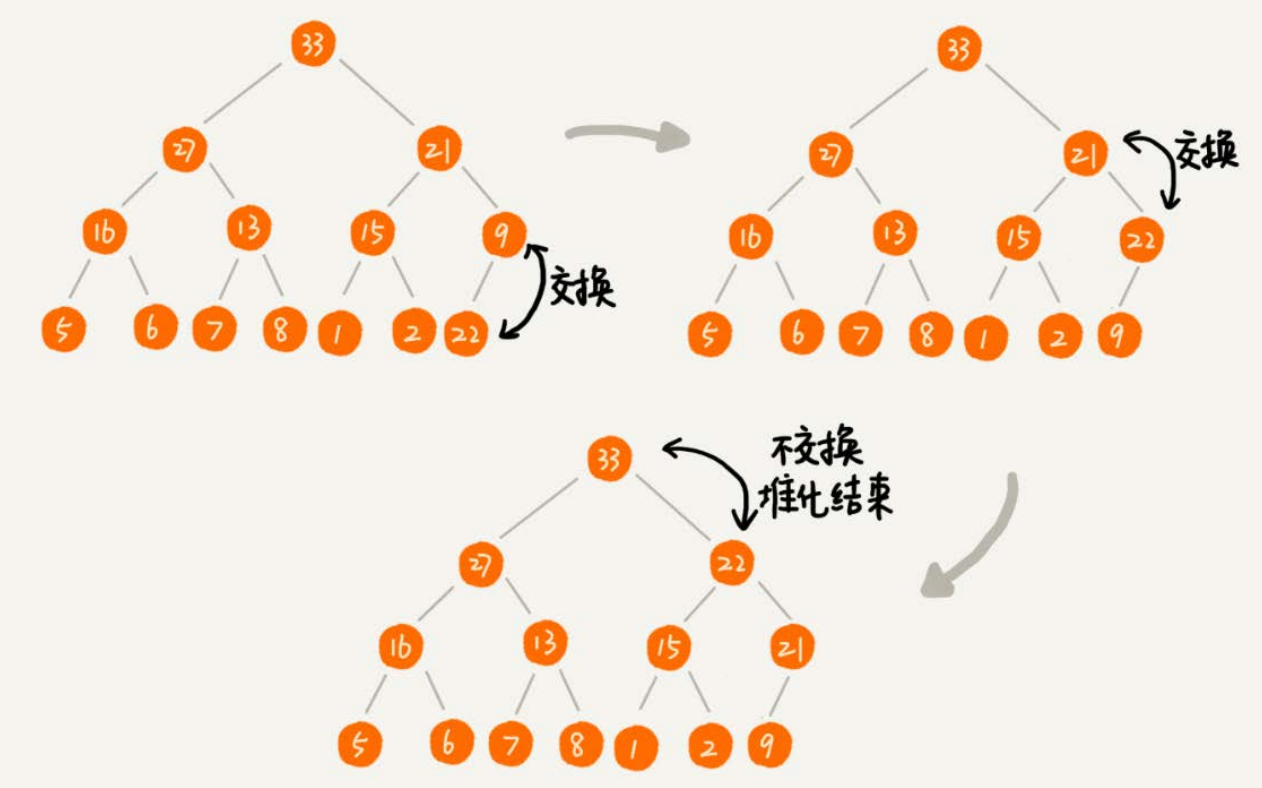public class Heap {
private int[] a; // 数组，从下标1开始存储数据
private int n; // 堆可以存储的最大数据个数
private int count; // 堆中已经存储的数据个数
public Heap(int capacity) {
a = new int[capacity + 1];
n = capacity;
count = 0;
}
public void insert(int data) {
if (count >= n) return; // 堆满了
++count;
a[count] = data;
int i = count;
while (i/2 > 0 && a[i] > a[i/2]) { // 自下往上堆化
swap(a, i, i/2); // swap()函数作用：交换下标为i和i/2的两个元素
i = i/2;
}
}
}

### 2.删除堆顶元素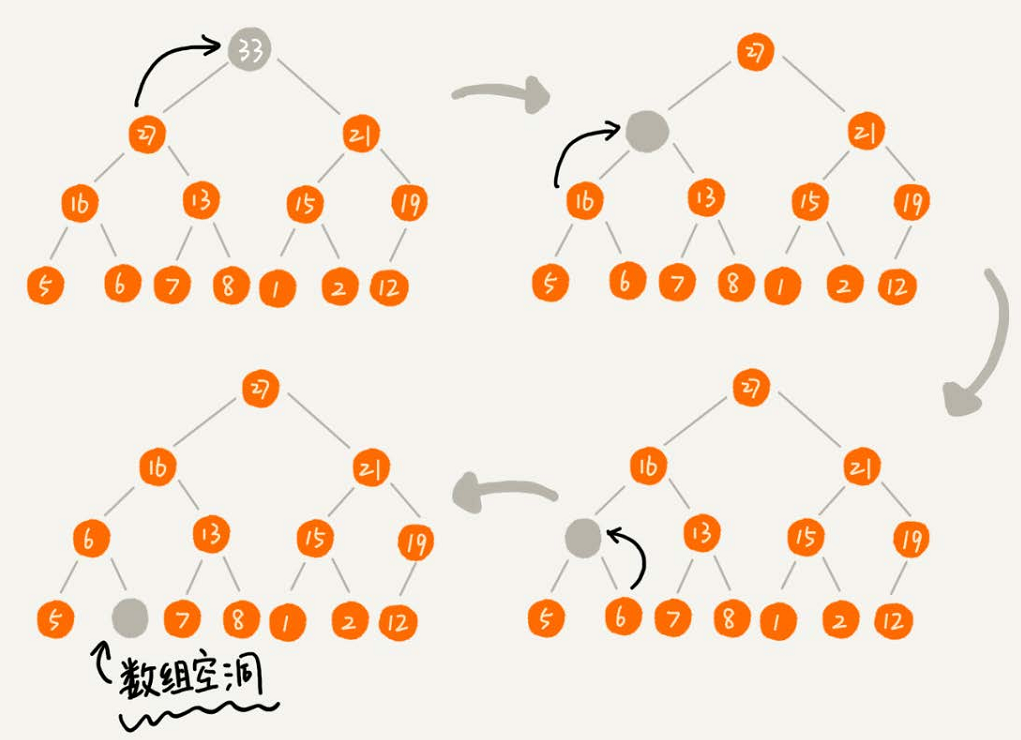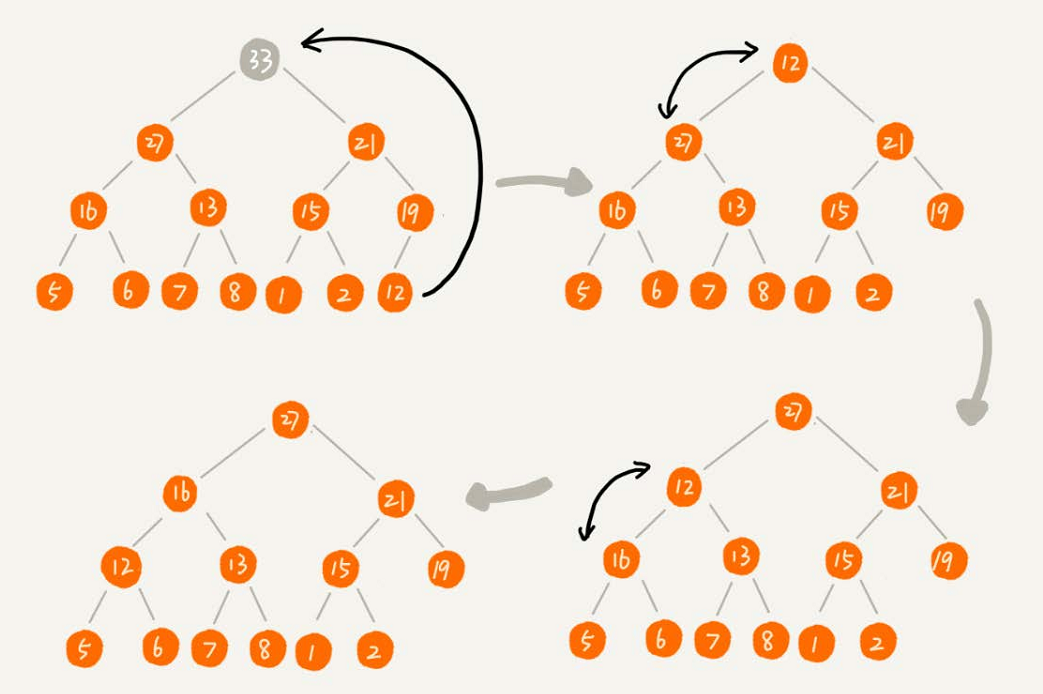public void removeMax() {
if (count == 0) return -1; // 堆中没有数据
a = a[count];
--count;
heapify(a, count, 1);
}
private void heapify(int[] a, int n, int i) { // 自上往下堆化
while (true) {
int maxPos = i;
if (i*2 <= n && a[i] < a[i*2]) maxPos = i*2;
if (i*2+1 <= n && a[maxPos] < a[i*2+1]) maxPos = i*2+1;
if (maxPos == i) break;
swap(a, i, maxPos);
i = maxPos;
}
}

### 如何基于堆实现排序？

1. 建堆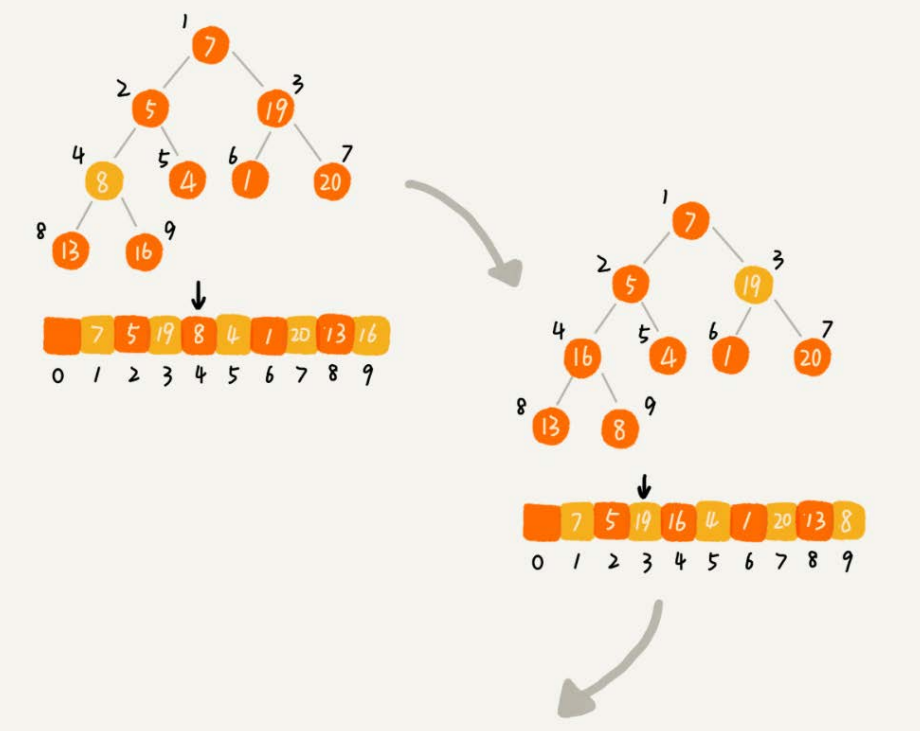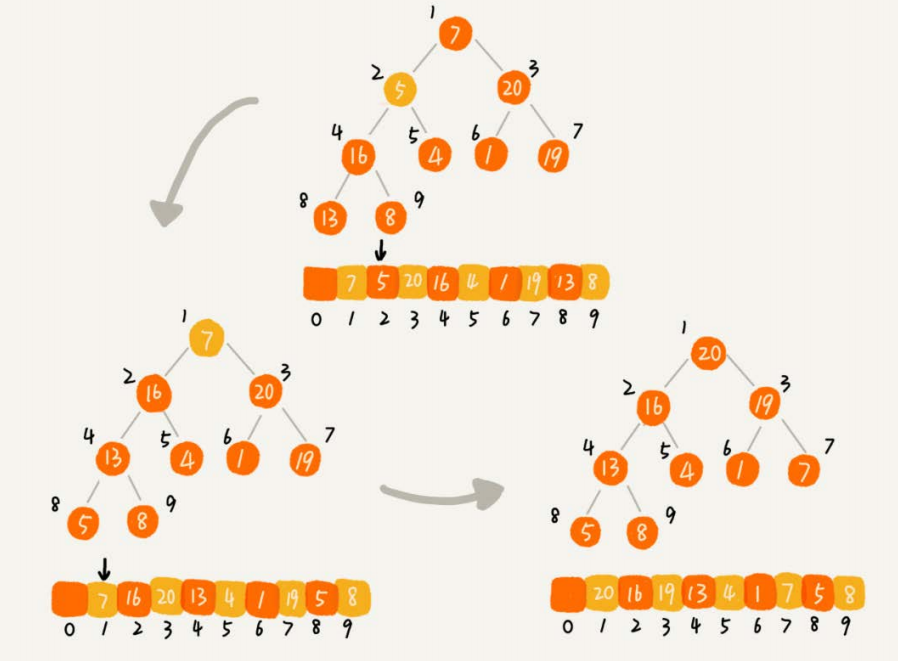private static void buildHeap(int[] a, int n) {
for (int i = n/2; i >= 1; --i) {
heapify(a, n, i);
}
}
private static void heapify(int[] a, int n, int i) {
while (true) {
int maxPos = i;
if (i*2 <= n && a[i] < a[i*2]) maxPos = i*2;
if (i*2+1 <= n && a[maxPos] < a[i*2+1]) maxPos = i*2+1;
if (maxPos == i) break;
swap(a, i, maxPos);
i = maxPos;
}
}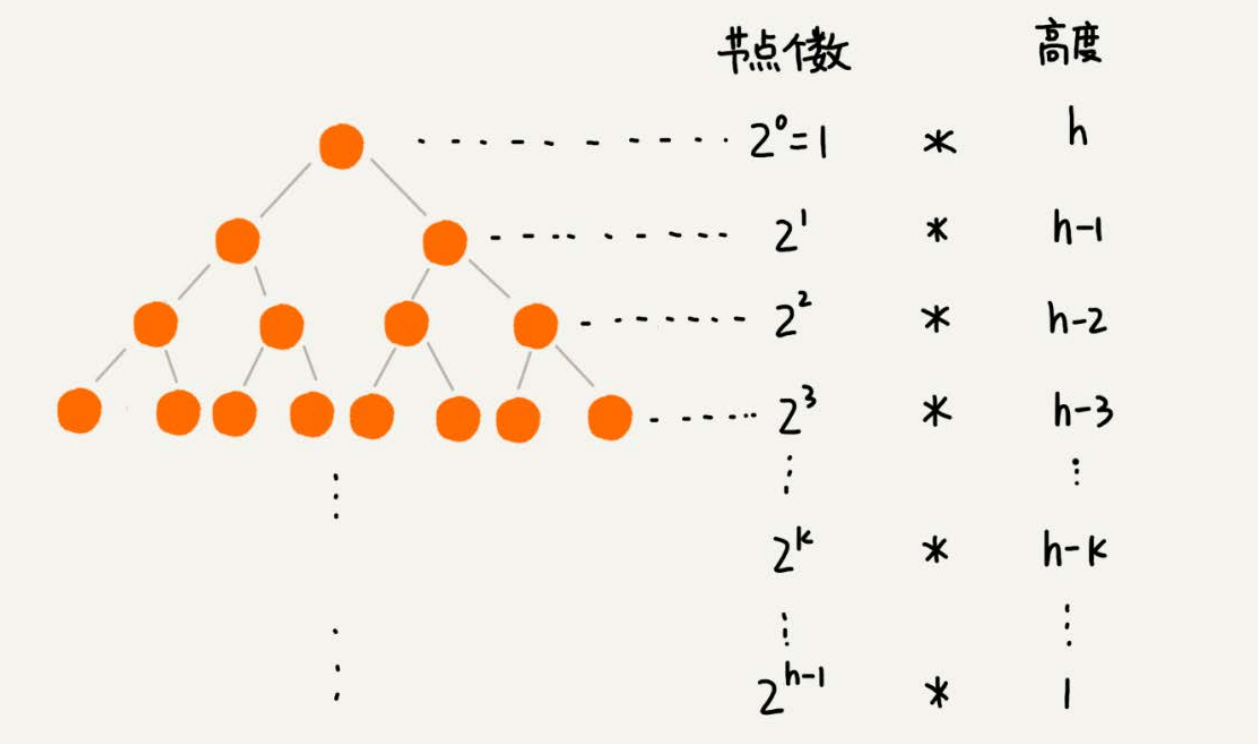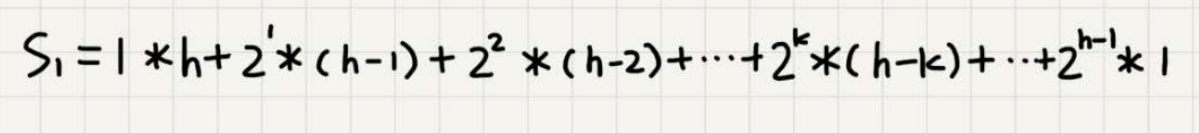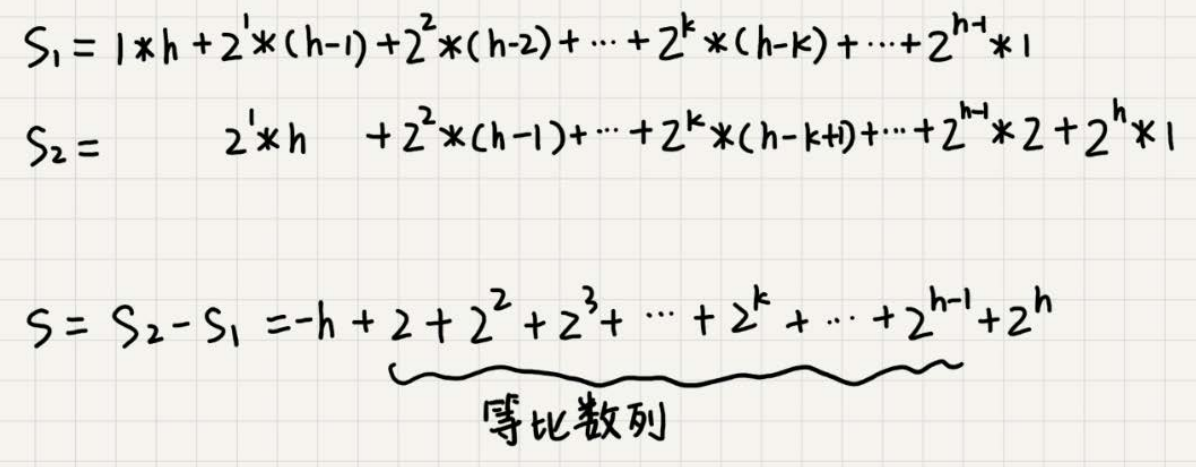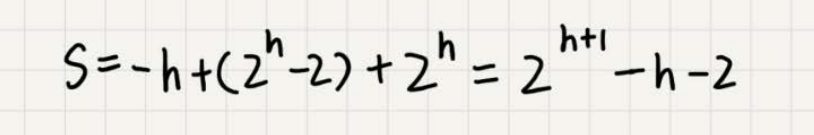2.排序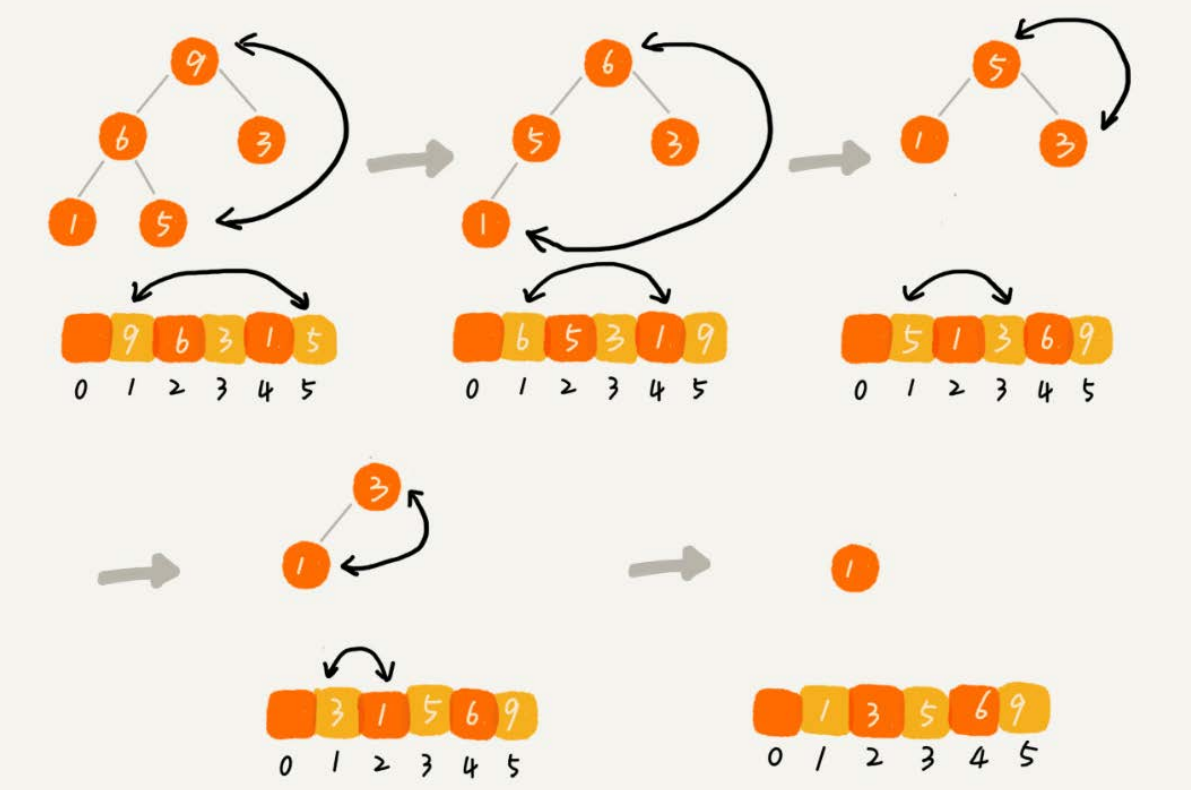// n表示数据的个数，数组a中的数据从下标1到n的位置。
public static void sort(int[] a, int n) {
buildHeap(a, n);
int k = n;
while (k > 1) {
swap(a, 1, k);
--k;
heapify(a, k, 1);
}
}

### 堆的应用除了堆排以外，还有如下一些应用：

1. 从大数量级数据中筛选出 top n 条数据； 比如：从几十亿条订单日志中筛选出金额靠前的 1000 条数据
2. 在一些场景中，会根据不同优先级来处理网络请求，此时也可以用到优先队列 ( 用堆实现的数据结构 ) ；比如：网络框架 Volley 就用了 Java 中PriorityBlockingQueue ，当然它是线程安全的
3. 可以用堆来实现多路归并，从而实现有序， leetcode 上也有相关的一题： Merge K Sorted Lists

## 二分查找

### O(logn)惊人的查找速度### 思考题

1. 如何编程实现“求一个数的平方根”？要求精确到小数点后6位。

int abs(int i);            处理int类型的取绝对值

double fabs(double i);     处理double类型的取绝对值

float fabsf(float i);      处理float类型的取绝对值

2. 如果数据使用链表存储，二分查找的时间复杂就会变得很高，那查找的时间复杂度究竟是多少呢？

......

## 二分查找的变体问题

### 变体一：查找第一个值等于给定值的元素

public int bsearch(int[] a, int n, int value) {
int low = 0;
int high = n - 1;
while (low <= high) {
int mid = low + ((high - low) >> 1);
if (a[mid] >= value) {     /注意这里是>=，因为要找的是第一个val值的下标
high = mid - 1;
} else {
low = mid + 1;
}
}
if (low < n && a[low]==value) return low;
else return -1;
}
public int bsearch(int[] a, int n, int value) {
int low = 0;
int high = n - 1;
while (low <= high) {
int mid = low + ((high - low) >> 1);
if (a[mid] > value) {   大于val
high = mid - 1;
} else if (a[mid] < value) {   小于val
low = mid + 1;
} else {   等于val
if ((mid == 0) || (a[mid - 1] != value)) return mid;    /是第一个元素 或 前面的元素不再=val
else high = mid - 1;    /前面还要=val的值
}
}
return -1;
}

### 变体二：查找最后一个值等于给定值的元素

public int bsearch(int[] a, int n, int value) {
int low = 0;
int high = n - 1;
while (low <= high) {
int mid = low + ((high - low) >> 1);
if (a[mid] > value) {
high = mid - 1;
} else if (a[mid] < value) {
low = mid + 1;
} else {
if ((mid == n - 1) || (a[mid + 1] != value)) return mid;
else low = mid + 1;   /区别在于这时候应该向右搜索
}
}
return -1;
}

### 变体三：查找第一个大于等于给定值的元素

public int bsearch(int[] a, int n, int value) {
int low = 0;
int high = n - 1;
while (low <= high) {
int mid = low + ((high - low) >> 1);
if (a[mid] >= value) {
if ((mid == 0) || (a[mid - 1] < value)) return mid;   /不用额外判断=val的情况
else high = mid - 1;
} else {
low = mid + 1;
}
}
return -1;
}

### 变体四：查找最后一个小于等于给定值的元素

public int bsearch7(int[] a, int n, int value) {
int low = 0;
int high = n - 1;
while (low <= high) {
int mid = low + ((high - low) >> 1);
if (a[mid] > value) {
high = mid - 1;
} else {
if ((mid == n - 1) || (a[mid + 1] > value)) return mid;  /这时包含在<=val的情况下
else low = mid + 1;
}
}
return -1;
}

# 跳表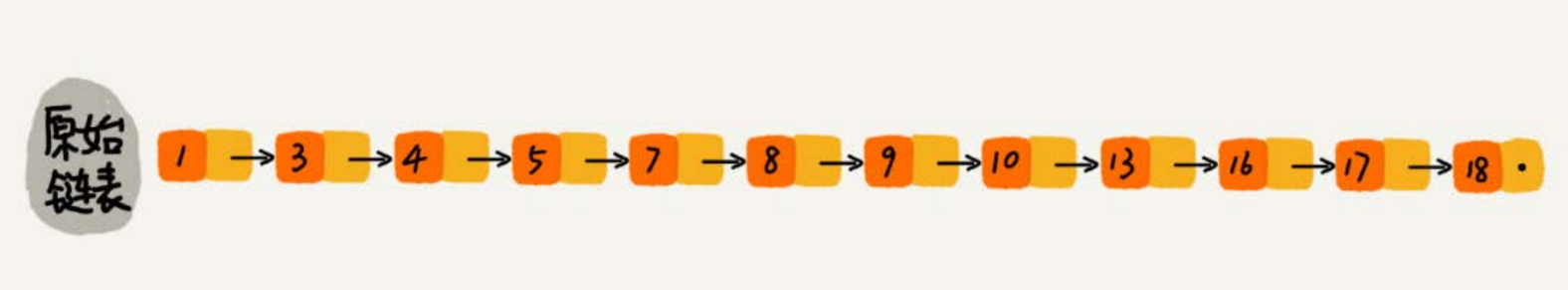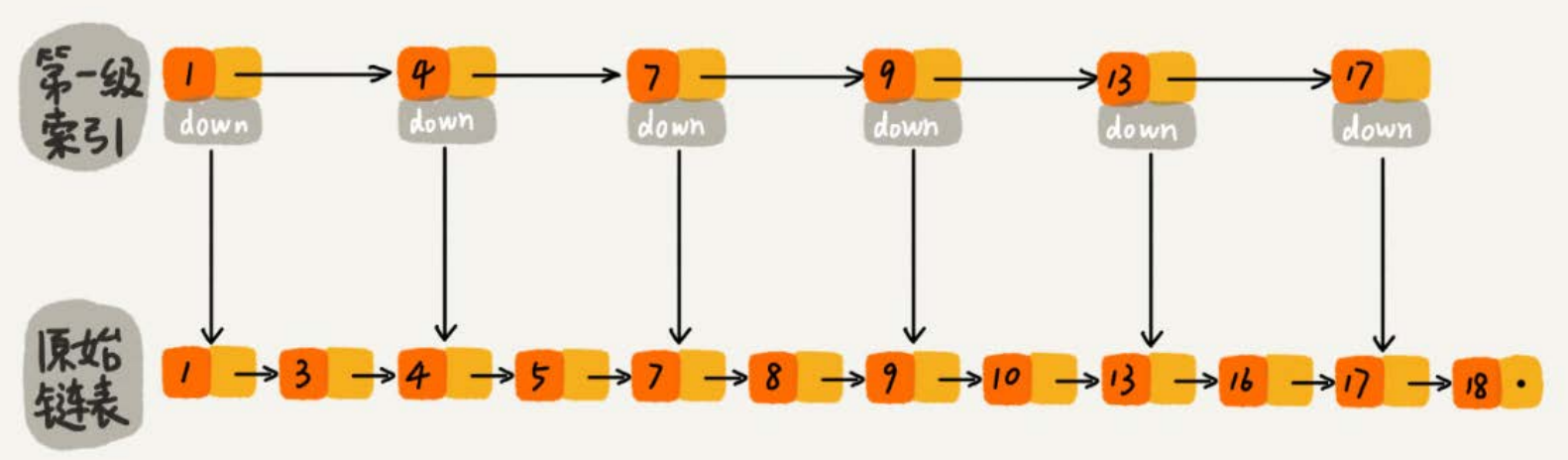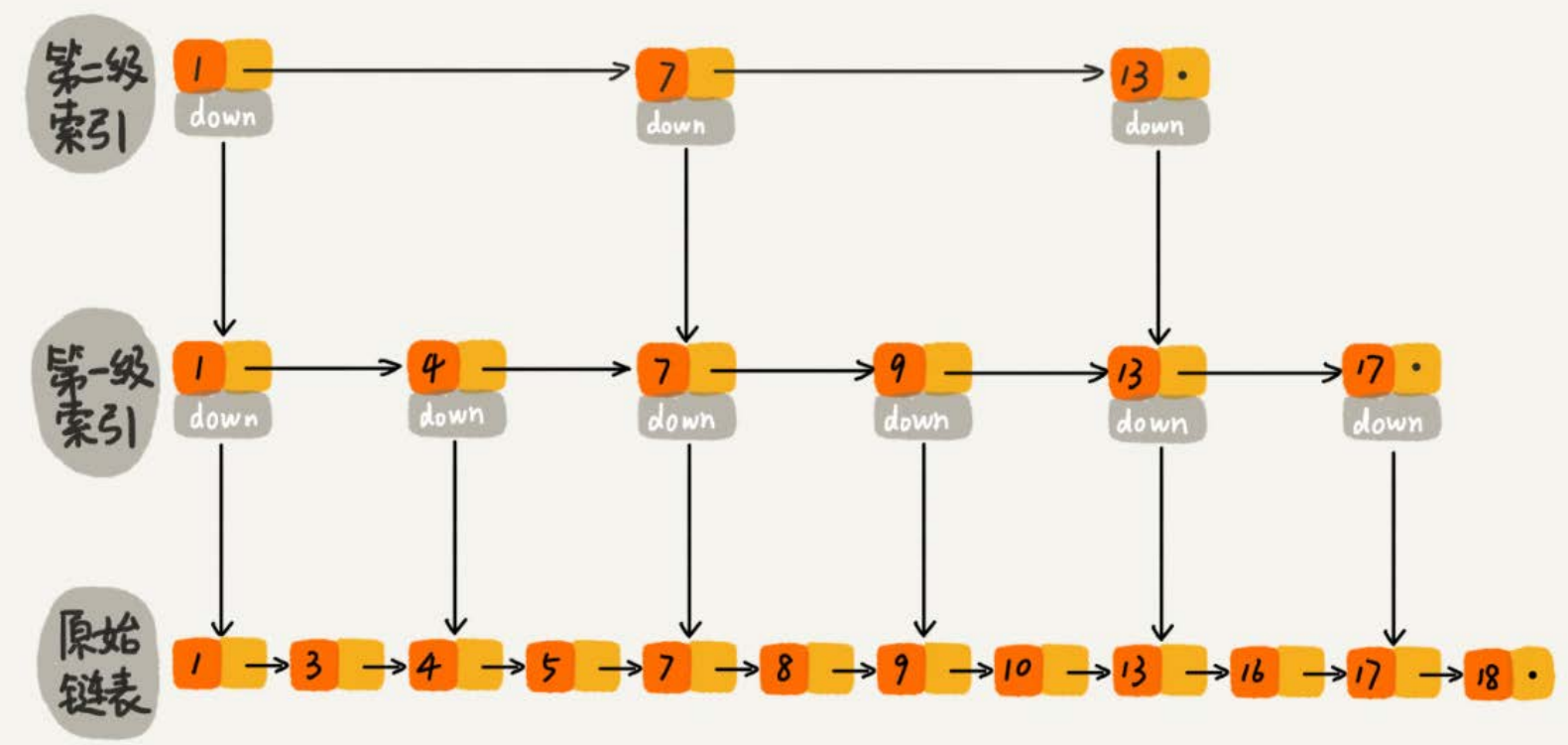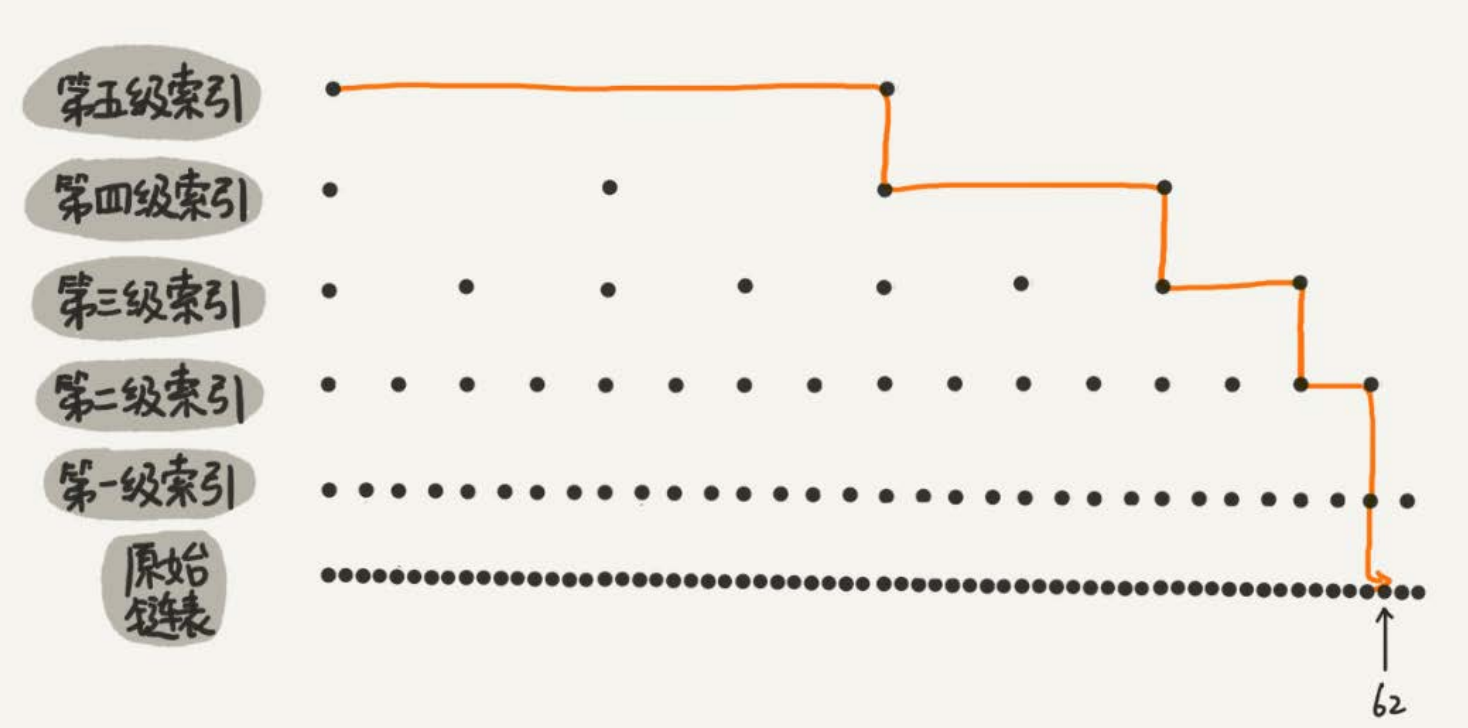### 用跳表查询到底有多快？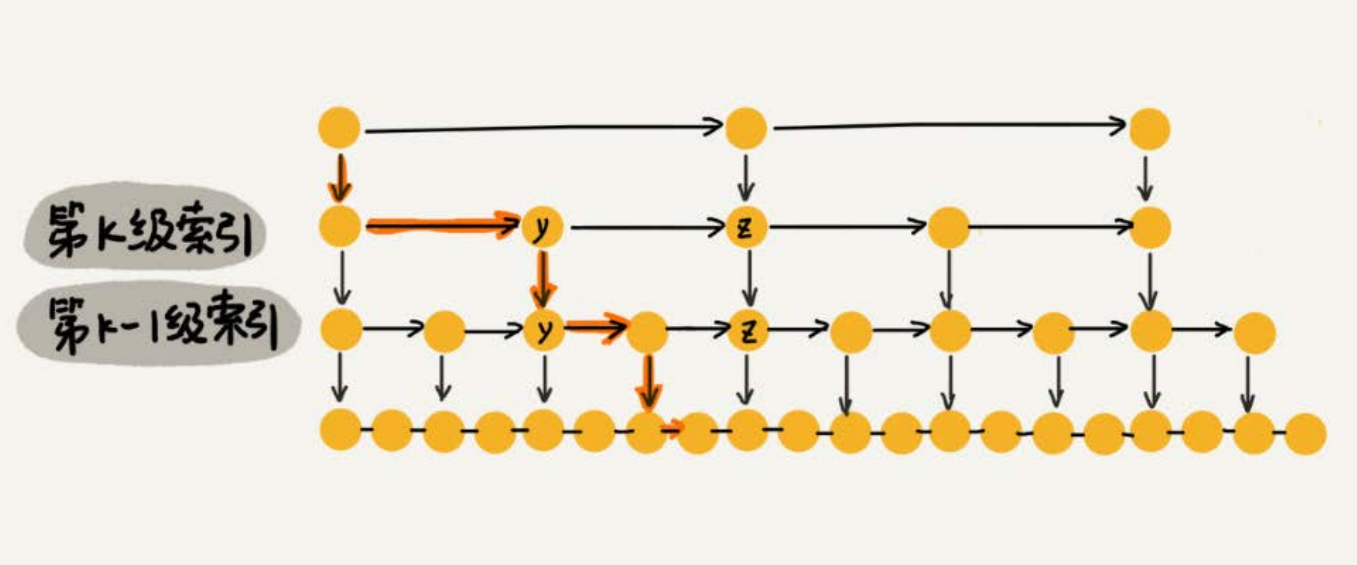### 跳表是不是很浪费内存？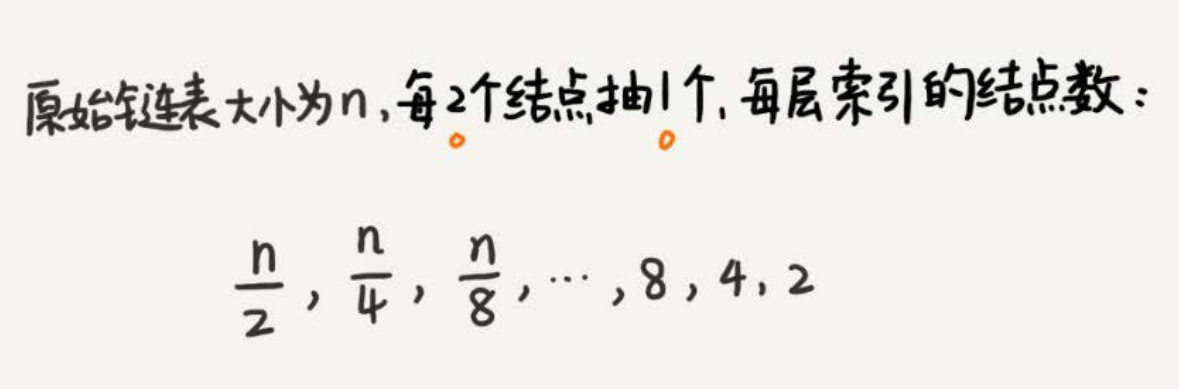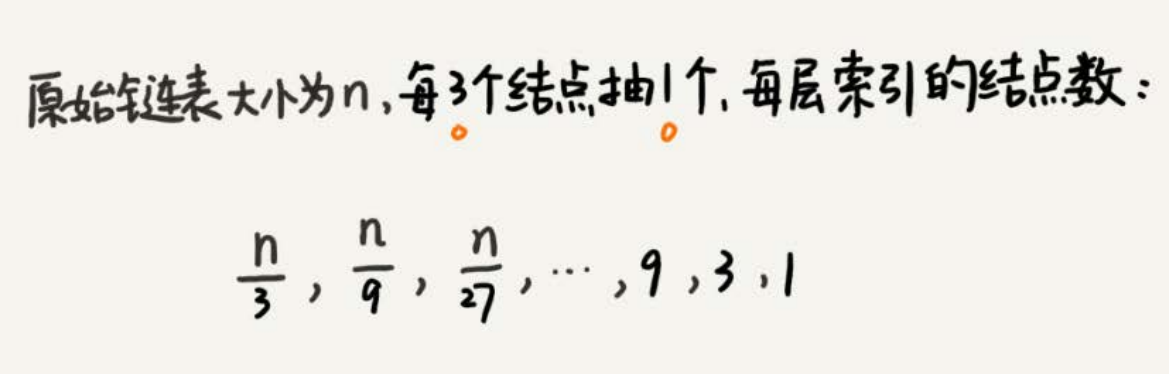### 为什么Redis要用跳表来实现有序集合，而不是红黑树？

Redis中的有序集合是通过跳表来实现的，严格点讲，其实还用到了散列表。Redis中的有序集合支持的核心操作主要有下面这几个：

• 插入一个数据；
• 删除一个数据；
• 查找一个数据；
• 按照区间查找数据（比如查找值在[100, 356]之间的数据）；
• 迭代输出有序序列。

## 散列表

### 散列冲突

1.开放寻址法

2.链表法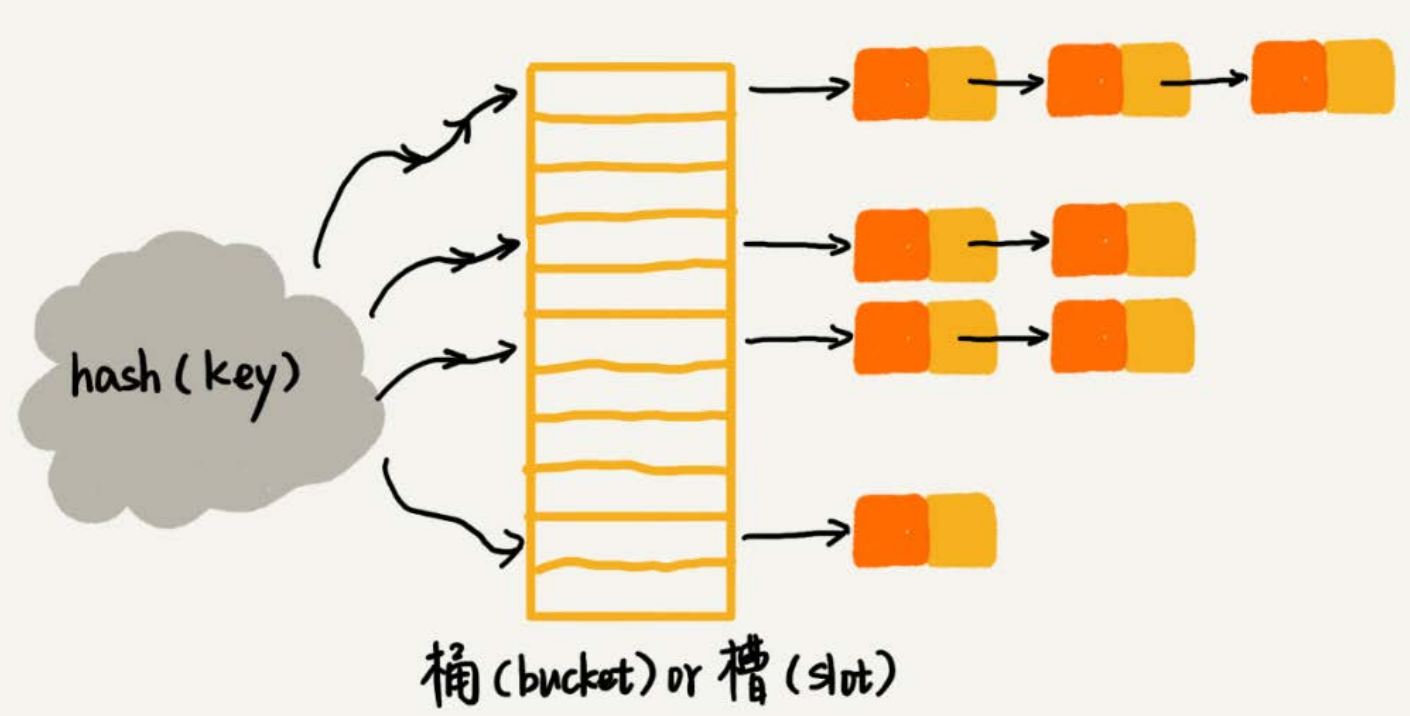### 思考题

1.Word文档中单词拼写检查功能是如何实现的？

2. 假设我们有10万条URL访问日志，如何按照访问次数给URL排序？

3. 有两个字符串数组，每个数组大约有10万条字符串，如何快速找出两个数组中相同的字符串？

### 如何设计散列函数？

hash("nice")=(("n" - "a") * 26*26*26 + ("i" - "a")*26*26 + ("c" - "a")*26+ ("e"-"a")) / 78978

1.开放寻址法

2.链表法

### 工业级散列表举例分析

1.初始大小

HashMap默认的初始大小是16，当然这个默认值是可以设置的，如果事先知道大概的数据量有多大，可以通过修改默认初始大小，减少动态扩容的次数，这样会大大提高HashMap的性能。

2.装载因子和动态扩容

3.散列冲突解决方法

HashMap底层采用链表法来解决冲突。即使负载因子和散列函数设计得再合理，也免不了会出现拉链过长的情况，一旦出现拉链过长，则会严重影响HashMap的性能。

4.散列函数

### 如何设计的一个工业级的散列函数？

• 支持快速的查询插入删除操作；
• 内存占用合理，不能浪费过多的内存空间；
• 性能稳定，极端情况下，散列表的性能也不会退化到无法接受的情况。

• 设计一个合适的散列函数
• 定义装载因子阈值，并且设计动态扩容策略
• 选择合适的散列冲突解决方法

### LRU缓存淘汰算法

• 往缓存中添加一个数据；
• 从缓存中删除一个数据；
• 在缓存中查找一个数据。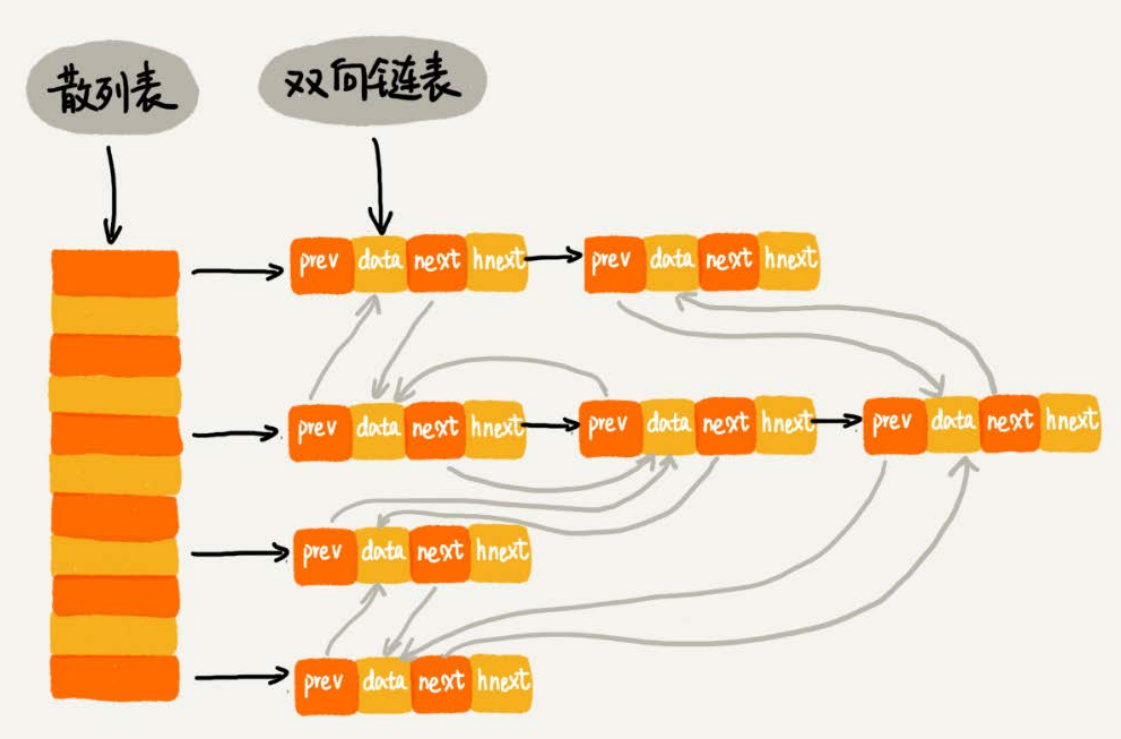### 什么是哈希算法？

• 从哈希值不能反向推导出原始数据（所以哈希算法也叫单向哈希算法）；
• 对输入数据非常敏感，哪怕原始数据只修改了一个Bit，最后得到的哈希值也大不相同；
• 散列冲突的概率要很小，对于不同的原始数据，哈希值相同的概率非常小；
• 哈希算法的执行效率要尽量高效，针对较长的文本，也能快速地计算出哈希值

### 数据校验

BT下载的原理是基于P2P协议的。我们从多个机器上并行下载一个2GB的电影，这个电影文件可能会被分割成很多文件块（比如可以分成100块，每块大约20MB）。等所有的文件块都下载完成之后，再组装成一个完整的电影文件就行了。

### 思考题

1.安全加密

①常用于加密的哈希算法：

MD5：MD5 Message-Digest Algorithm，MD5消息摘要算法

SHA：Secure Hash Algorithm，安全散列算法

DES：Data Encryption Standard，数据加密标准

②对用于加密的哈希算法，有两点格外重要，第一点是很难根据哈希值反向推导出原始数据，第二点是散列冲突的概率要小。

③在实际开发中要权衡破解难度和计算时间来决定究竟使用哪种加密算法。

2.唯一标识

3.数据校验

4.散列函数

### 哈希算法在分布式系统中的应用

1.负载均衡

1.1.需求

1.2.解决方案

2.数据分片

2.1.如何统计“搜索关键词”出现的次数？

①需求描述

②问题分析

③解决方案

2.2.如何快速判断图片是否存在图库中？

①需求描述

②问题分析

②解决方案

3.分布式存储

3.1.什么是分布式存储？

3.2.遇到的问题是什么？

3.3.解决方案是什么？

①这时，需要一种方法，使得新加入一个机器后，并不需要做大量的数据搬移。那就是在分布式系统中应用非常广泛的一致性哈希算法。

②一致性哈希算法的基本思想是什么呢？为了说清楚这个问题，我们假设有k个机器，数据的哈希值范围是[0-MAX]，我们将整个范围划分成m个小区间（m远大于k），每个机器复杂m/k个小区间。当有新机器加入的时候，我们就将某几个小区间的数据，从原来的机器中搬移到新的机器中。这样，既不用全部重新哈希、搬移数据，也保持了各个机器上数据量的均衡。

# 树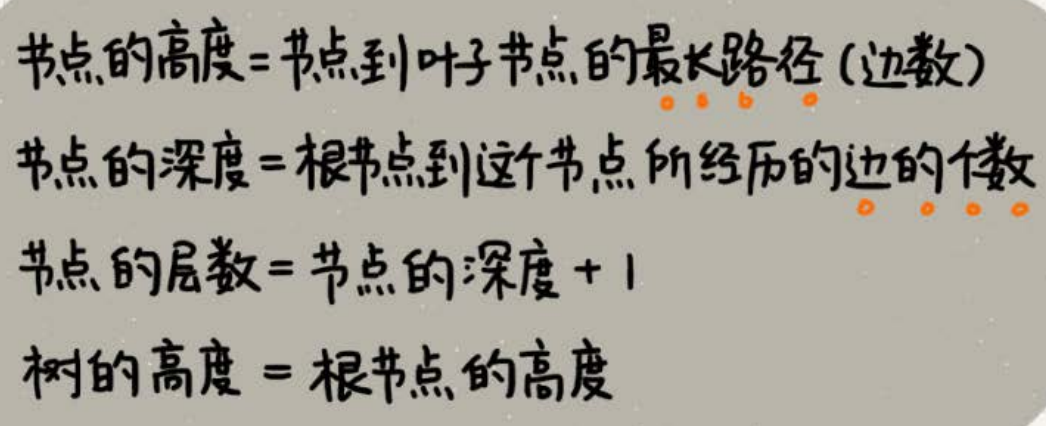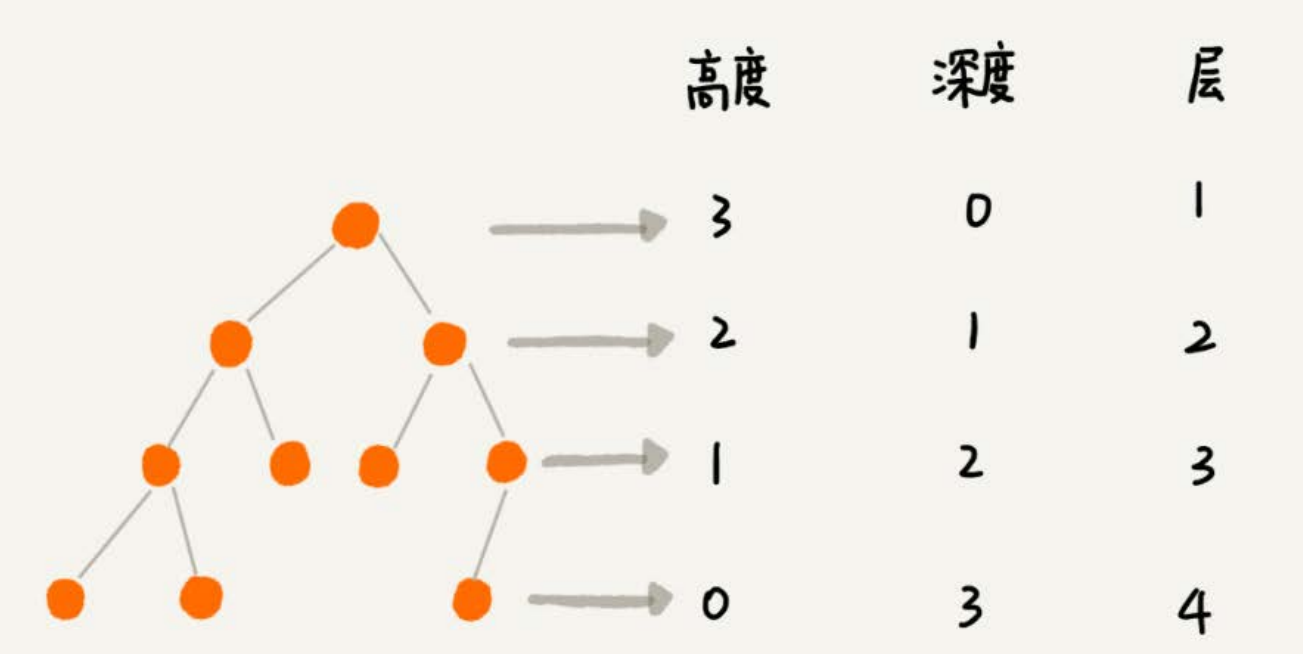# 二叉树（Binary Tree）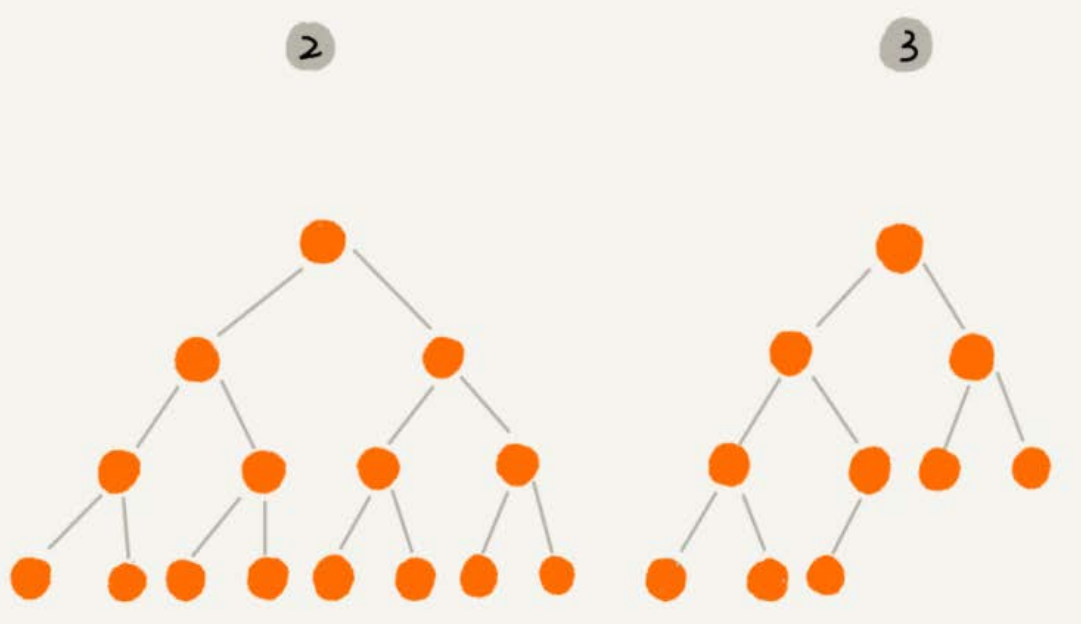### 二叉树的遍历

• 前序遍历是指，对于树中的任意节点来说，先打印这个节点，然后再打印它的左子树，最后打印它的右子树。
• 中序遍历是指，对于树中的任意节点来说，先打印它的左子树，然后再打印它本身，最后打印它的右子树。
• 后序遍历是指，对于树中的任意节点来说，先打印它的左子树，然后再打印它的右子树，最后打印这个节点本身。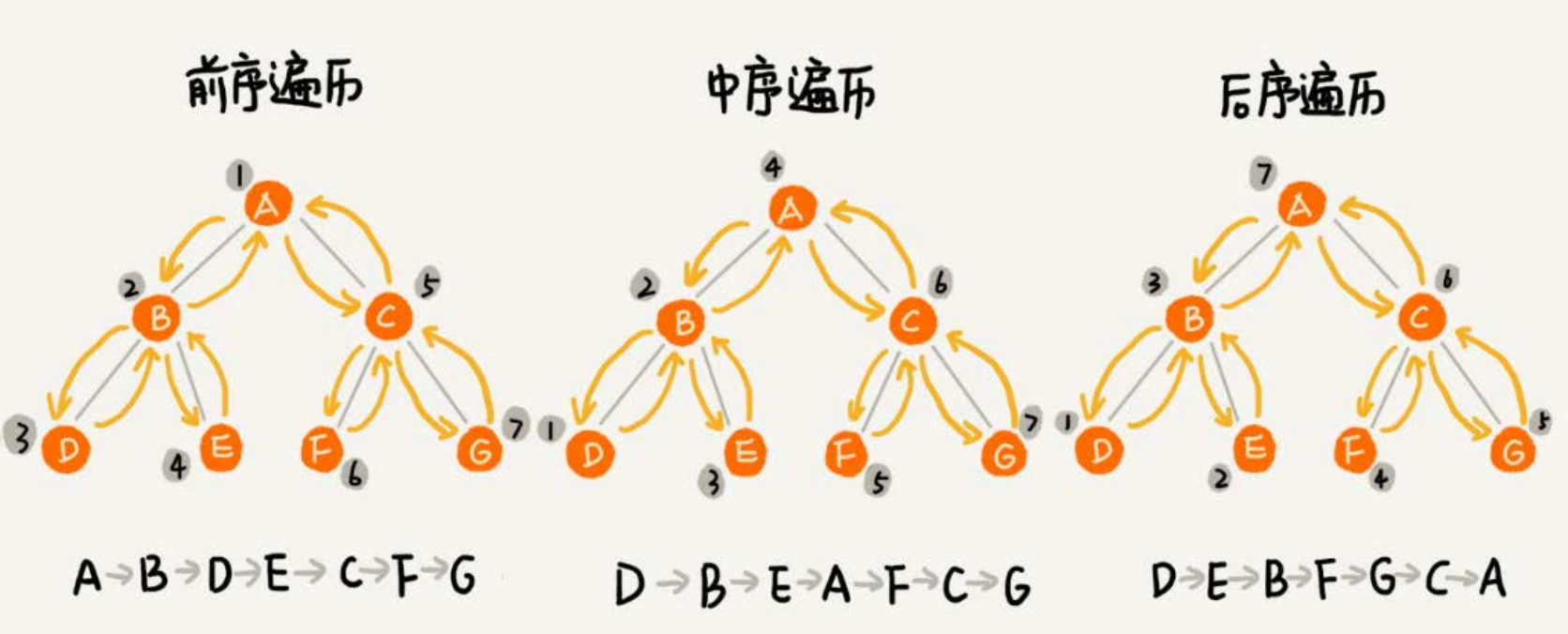## 二叉查找树（Binary Search Tree）

1.二叉查找树的查找操作

2.二叉查找树的插入操作

3.二叉查找树的删除操作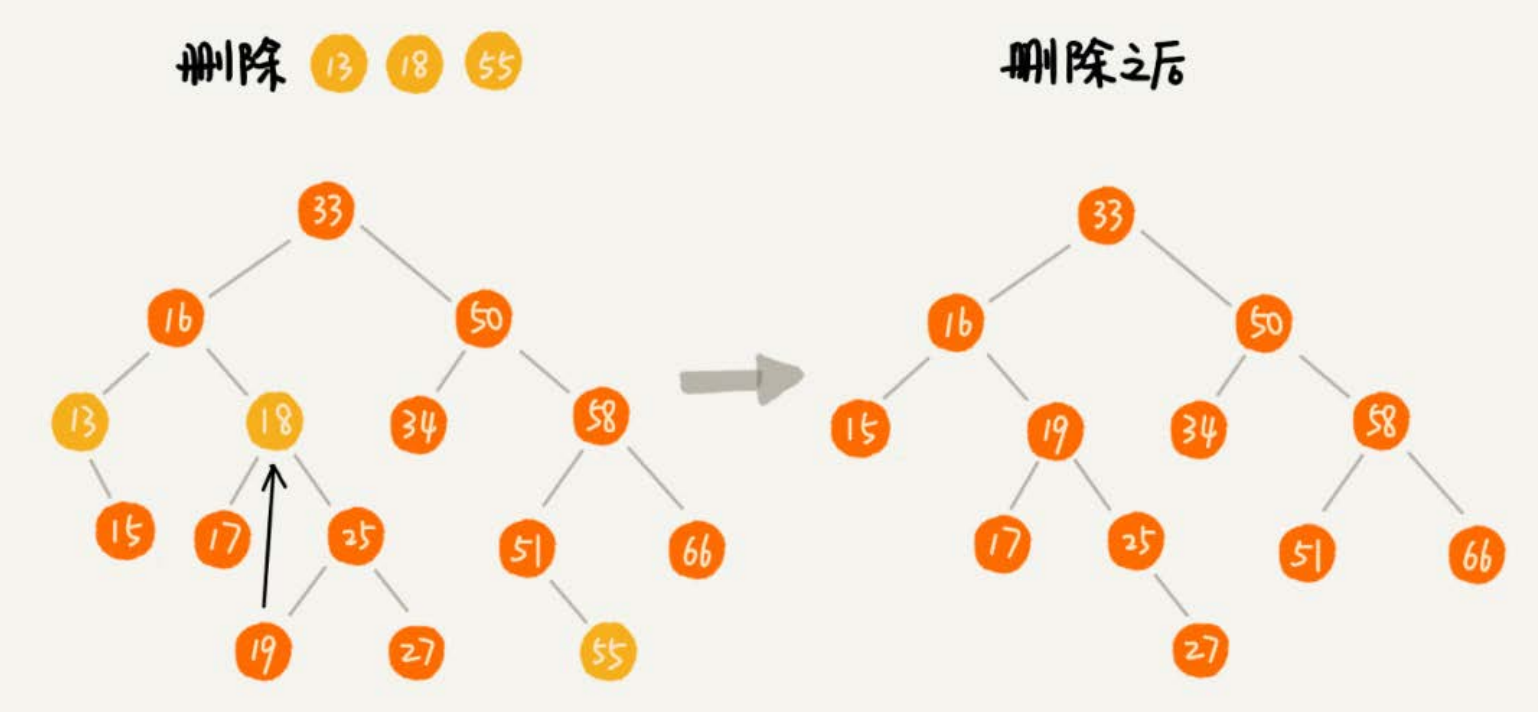public void delete(int data) {
Node p = tree; // p指向要删除的节点，初始化指向根节点
Node pp = null; // pp记录的是p的父节点
while (p != null && p.data != data) {
pp = p;
if (data > p.data) p = p.right;
else p = p.left;
}
if (p == null) return; // 没有找到
if (p.left != null && p.right != null) { // 查找右子树中最小节点
Node minP = p.right;
Node minPP = p; // minPP表示minP的父节点
while (minP.left != null) {
minPP = minP;
minP = minP.left;
}
p.data = minP.data; // 将minP的数据替换到p中
p = minP; // 下面就变成了删除minP了
pp = minPP;
}
// 删除节点是叶子节点或者仅有一个子节点
Node child; // p的子节点
if (p.left != null) child = p.left;
else if (p.right != null) child = p.right;
else child = null;
if (pp == null) tree = child; // 删除的是根节点
else if (pp.left == p) pp.left = child;
else pp.right = child;
}

### 支持重复数据的二叉查找树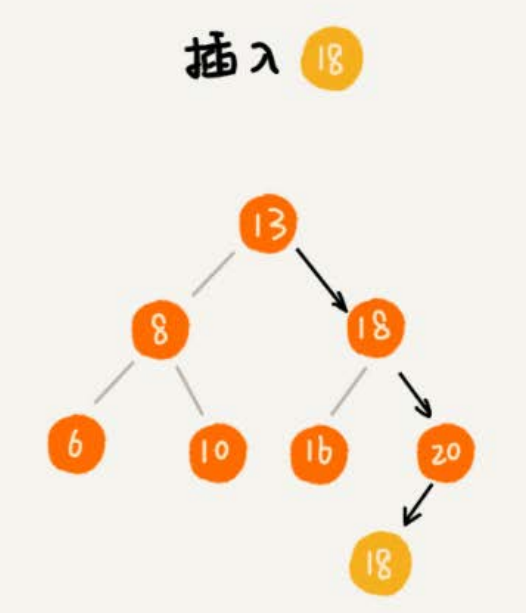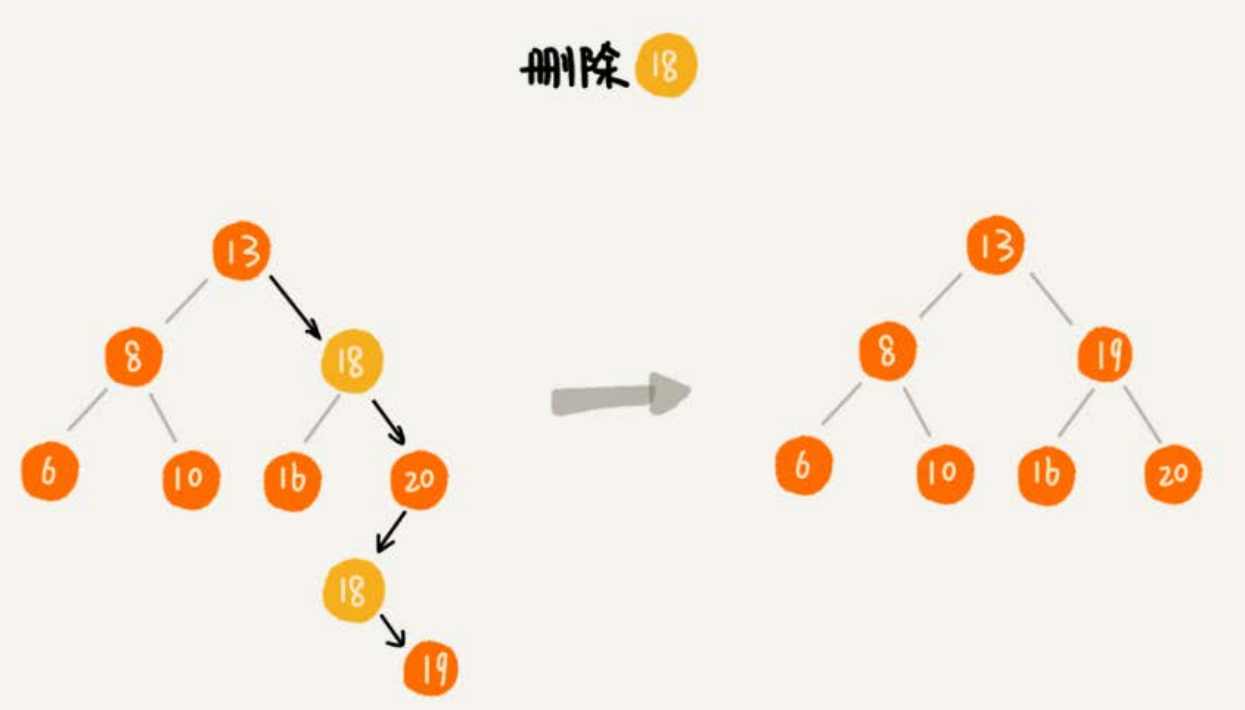### 二叉查找树的时间复杂度分析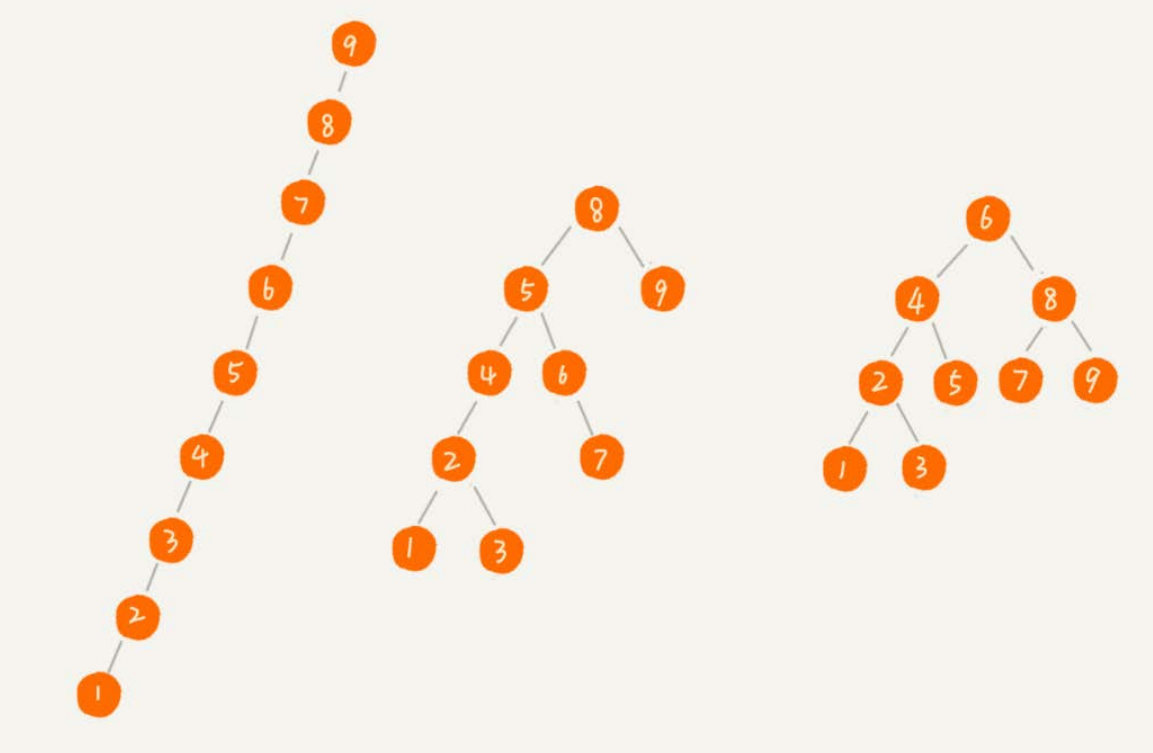n >= 1+2+4+8+...+2^(L-2)+1

n <= 1+2+4+8+...+2^(L-2)+2^(L-1)

# 红黑树（不必细抠实现，理解思路应该差不多了）

## 什么是“平衡二叉查找树”？

发明平衡二叉查找树这类数据结构的初衷是，解决普通二叉查找树在频繁的插入、删除等动态更新的情况下，出现时间复杂度退化的问题。

## 如何定义一棵“红黑树”？

• 根节点黑色的；
• 每个叶子节点都是黑色空节点（NIL），也就是说，叶子节点不存储数据
• 任何相邻的节点不能同时为红色，也就是说，红色节点是被黑色节点隔开的；
• 每个节点，从该节点到达其可达叶子节点所有路径，都包含相同数目黑色节点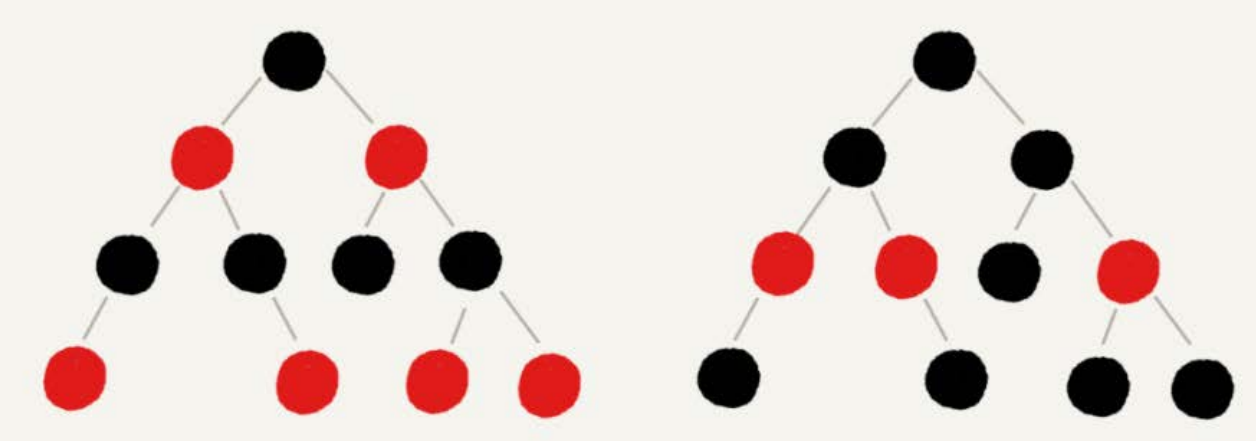### 为什么说红黑树是“近似平衡”的？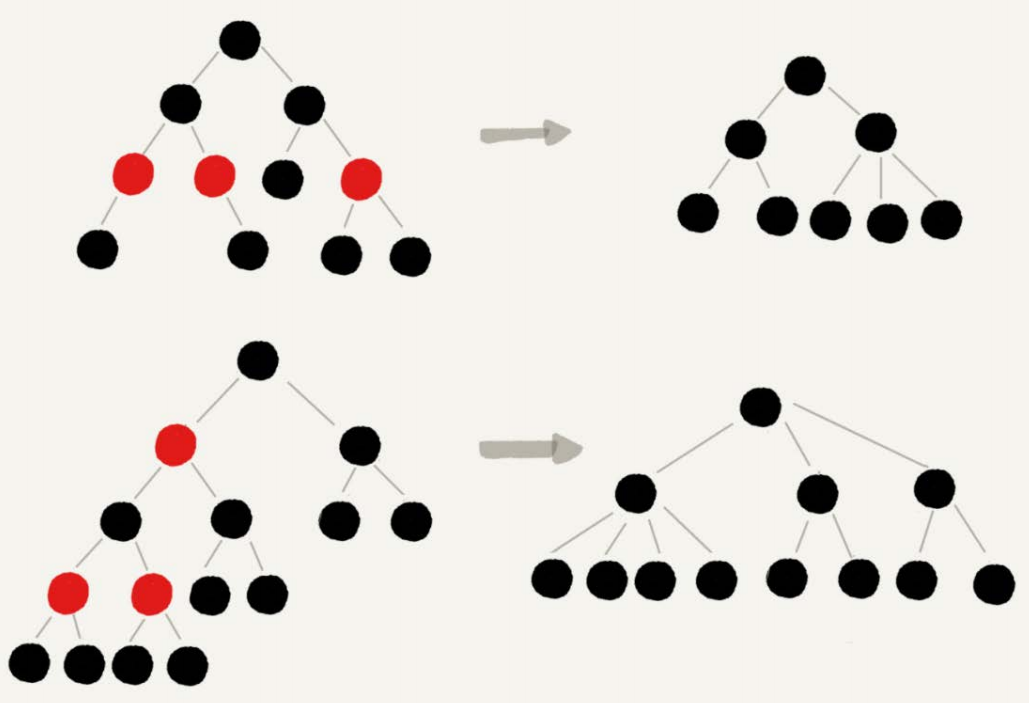## 为什么在工程中大家都喜欢用红黑树这种平衡二叉查找树？

AVL树是一种高度平衡的二叉树，所以查找的效率非常高，但是，有利就有弊，AVL树为了维持这种高度的平衡，就要付出更多的代价。每次插入、删除都要做调整，就比较复杂、耗时。所以，对于有频繁的插入、删除操作的数据集合，使用AVL树的代价就有点高了。

03-1116012-03275
06-04802
04-19256
10-11154
07-07542
02-2463
05-15356
11-187508